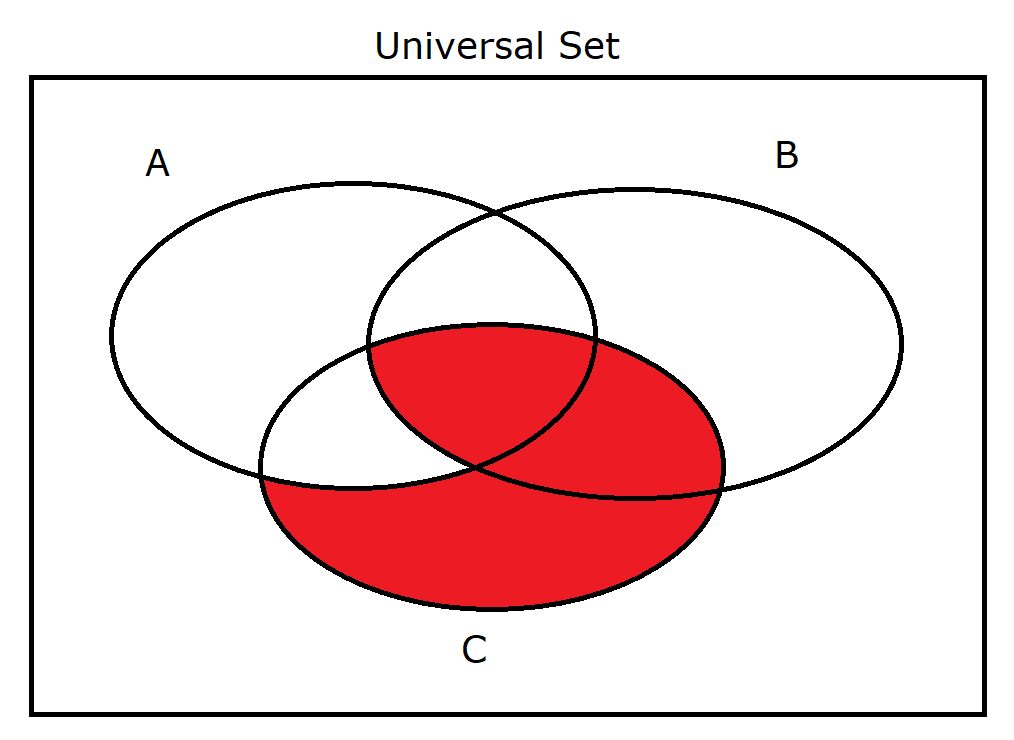If there is one prayer that you should pray/sing every day and every hour, it is the LORD's prayer (Our FATHER in Heaven prayer)
It is the most powerful prayer. A pure heart, a clean mind, and a clear conscience is necessary for it.
- Samuel Dominic Chukwuemeka

For in GOD we live, and move, and have our being. - Acts 17:28

The Joy of a Teacher is the Success of his Students. - Samuel Dominic Chukwuemeka

# Welcome to Set TheoryI greet you this day,
Second: view the videos.
Third: solve the questions/solved examples.
Fourth: check your solutions with my thoroughly-explained solutions.
Comments, ideas, areas of improvement, questions, and constructive criticisms are welcome. You may contact me.
If you are my student, please do not contact me here. Contact me via the school's system. Thank you for visiting.

Samuel Dominic Chukwuemeka (Samdom For Peace) B.Eng., A.A.T, M.Ed., M.S

## Set Theory

### Objectives

Students will:

(1.) Define sets.
(2.) Discuss the terms used in sets.
(3.) Determine the union of sets.
(4.) Determine the intersection of sets.
(5.) Determine the complement of a set.
(6.) List the subsets of a set.
(7.) List the power set of a set.
(8.) Determine the difference of sets.
(9.) Determine the symmetric difference of sets.
(10.) Determine the Cartesian product of sets.
(11.) Draw Venn diagrams for two sets.
(12.) Draw Venn diagrams for three sets.
(13.) Solve applied problems involving two sets.
(14.) Solve applied problems involving three sets.

Have you ever used the word, "Set"? In what way(s) did you use it?

### Why Study Sets?

Sets are used in:
Political applications - in polls and surveys among others.

If there is a large class, do some "fun applications" of sets.

### Definitions

Set theory is the branch of mathematics that deals with the study of sets.

A Set is a collection of things such as items and objects among others.
It is denoted by an upper-case letter, say $A$.
Sets can be well-defined sets or non well-defined sets.

A Well-defined set is a set arranged in order.
It does not depend on the opinion of the individual.
It is not biased.

Examples are:
the set of computer science books at the AWC library arranged by their dates of publications
the set of students in Mr. C's class arranged in alphabetical order of their last names

A Non well-defined set is a set that depends on the opinion of the individual.
It is biased.

Examples are:
the set of the three best movies that were released last year
the set of the five most handsome guys at AWC. If you do not include me in the set, then it is a wrong set ☺☺☺

We shall only deal with Well-defined sets

We also have finite sets and infinite sets.

A Finite set is a set that has a finite number of elements.
It has a bound.
It may have no elements or a positive integer number of elements.

An Infinite set is a set that is not finite.

We shall only deal with Finite sets

### Description of Sets

A set can be written or described in three ways. They are:

(1.) Description: This method describes the sets in words.

(2.) Roster Form: This method uses a roster (similar to a classroom roster) to describe sets.
The roster form uses braces.
The elements of the set are listed in a roster format, separated with commas.
If the roster is too long, ellipsis (three dots) are used to indicate continuation.

(3.) Set Notation: This method uses a "shortened" form to describe sets.
It is also known as Set Builder Notation or Set Generator Notation.
The set notation also uses braces.

Exercise 1
Assume Set $A$ contains the elements: Sunday, Monday, Tuesday, Wednesday, Thursday, Friday, and Saturday.
Describe set A using the three methods of describing sets.

Description: Set $A$ is the days of the week.
Roster Form: $A$ = {Sunday, Monday, Tuesday, ..., Friday, Saturday}
Set Notation: $A$ = {$x | x\:\: is\:\: a\:\: day\:\: of\:\: the\:\: week$}

## Symbols, Terms, and Definitions

Symbols Terms Definitions
(1.) $\{$ Set of $A$ = {... is read as: $A$ is a set of ...
(2.) $x |$ or $x:$ $x$ such that... $x | ...$ is read as: $x$ such that ...
(3.) $...$ Ellipsis This means continuation. It has to be only three dots.
(4.) $\infty$ Infinity Without bounds.
(5.) $\mu$ Universal Set It is the set that contains all the elements in a particular context.
It contains all the elements under consideration.
Every set is a subset of the universal set.
(6.) $phi$ or $\{\}$ Empty Set or Null Set It is a set that has no elements.
The cardinality of an empty set is zero.
Student: What is the cardinality of a set?
Teacher: It is the number of elements in the set.
It is also known as the cardinal number of a set.
There are no elements in an empty set.
Hence, the cardinality of a null set is $0$
NOTE: The null set is not $0$
It is the cardinality of the null set that is $0$
(7.) $\in$ element of or member of Say $A$ is a set of the vowels of the English language alphabets.
$A$ = $\{a, e, i, o, u\}$
$a\:\:\: \in\:\:\: A$ is read as $a$ is an element of Set $A$
$e\:\:\: \in\:\:\: A$
$i\:\:\: \in\:\:\: A$
$o\:\:\: \in\:\:\: A$
$u\:\:\: \in\:\:\: A$
(8.) $n$ Cardinality or Cardinal Number This is the number of elements of a set.
$n(A)$ is read as: the cardinality of Set $A$
It is the number of elements in Set $A$
(9.) $=$ Equals or Equal to $A = B$ is read as: Set $A$ is equal to Set $B$
Two sets are equal if they have the same elements, regardless of the order of arrangement of the elements.
(10.) $\equiv$ Equivalent $A \equiv B$ is read as: Set $A$ is equivalent to Set $B$
Two sets are equivalent if they have the same cardinality.
This means that they have the same number of elements.
(11.) $A'$ or $A^c$ Prime or Complement $A'$ or $A^c$ is read as: $A$-prime or $A$-complement
The complement of Set $A$ is the set that contains all the elements in the Universal set but are not in Set $A$.
You can see it as "Not $A$"
They do not like Set $A$ at all.
(12.) $\cup$ Union $A \cup B$ is read as: $A\:\: union\:\: B$
Look at it as "marriage".
The man brings what he has. Write it.
The woman brings what she has. Write it.
If they have anything in common, write it one time.

It is the set that contains:
all the elements of set $A$, OR
all the elements of set $B$, OR
all the elements of both sets $A$ AND $B$
(13.) $\cap$ Intersect $A \cap B$ is read as: $A\:\: intersect\:\: B$
Look at it as "intersection", "meet", "common".
It is the set that contains the elements in both sets $A$ AND $B$
(14.) $\subset$ Proper Subset $A \subset B$ is read as: Set $A$ is a proper subset of Set $B$
Look at it as "less than"
$$1 \lt 3 \\[2ex] 2 \lt 3$$

A set, say $A$ is a proper subset of another set, say $B$ if:
all the elements of Set $A$ are also in Set $B$ AND
Set $B$ contains at least one element that is not in Set $A$
(15.) $\supset$ Superset $B \supset A$ is read as: Set $B$ is a superset of Set $A$
Look at it as "greater than"
$$3 \gt 1 \\[2ex] 3 \lt 2$$

A set, say $B$ is a superset of another set, say $A$ if:
all the elements of Set $A$ are also in Set $B$ AND
Set $B$ contains at least one element that is not in Set $A$

In other words;
If Set A is a proper subset of Set $B$, then
Set $B$ is a superset of Set $A$
If $A \subset B$, then $B \supset A$
(16.) $\subseteq$ Subset $A \subseteq B$ is read as: Set $A$ is a subset of Set $B$
Look at it as "less than or equal to"
$$1 \le 3 \\[2ex] 2 \le 3 \\[2ex] 3 \le 3$$

A set, say $A$ is a subset of another set, say $B$ if:
all the elements of Set $A$ are also in Set $B$ AND
Set $B$ contains at least one element that is not in Set $A$
OR
all the elements of Set $B$ are also in Set $A$

Do you understand the difference between "less than" and "less than or equal to"
Do you understand the difference between "proper subset" and "subset"?

Subsets includes proper subsets and equal sets
(17.) $P$ Power Set $P(A)$ is read as: Power Set of Set $A$
The power set of a set is the set of all the subsets of the set.
The Power Set of Set $A$, $P(A)$ is the set of all the subsets of Set $A$
(18.) $A - B$ Difference of Sets $A - B$ is read as: Set $A$ difference Set $B$
Set $A$ difference Set $B$ is the set of elements in Set $A$, but not in Set $B$
(19.) $A \dot{-} B$ Symmetric Difference $A \dot{-} B$ is read as: Set $A$ symmetric difference Set $B$
Set $A$ symmetric difference Set $B$ is the set of elements in Set $A$ or Set $B$, but not in both Sets $A$ and $B$
(20.) $A \times B$ Cartesian Product $A \times B$ is read as: Set $A$ cross Set $B$
Set $A$ cross Set $B$ is the set of all possible ordered pairs of the form, $(a, b)$ where $a \in A$ and $b \in B$

## Set Algebra

### Power Set

The power set of a set, say $A$ is the set of all the subsets of set $A$.
It is denoted by $P(A)$

Given: Set $A$ with cardinality, $n$
$n(P(A)) = 2^n \\[3ex] n(Subsets) = 2^n \\[3ex] n(Proper\:\: Subsets) = 2^n - 1$

Exercise 2
For the Set $A = \{L, O, V, E\}$; determine:
(1.) The cardinality of set $A$
(2.) The set of the proper subsets.
(3.) The Power set (set of the subsets).
(4.) The cardinality of the proper subsets.
(5.) The cardinality of the power set.

(1.) $n(A) = 4$

(2.) The set of the proper subsets is:
{$\phi$, {L}, {O}, {V}, {E}, {L, O}, {L, V}, {L, E}, {O, V}, {O, E}, {V, E}, {L, O, V}, {L, O, E}, {L, V, E}, {O, V, E}}

(3.) The power set is, $P(A)$ is:
{$\phi$, {L}, {O}, {V}, {E}, {L, O}, {L, V}, {L, E}, {O, V}, {O, E}, {V, E}, {L, O, V}, {L, O, E}, {L, V, E}, {O, V, E}, {L, O, V, E}}

(4.) $n(Proper\:\: Subsets) = 15$

(5.) $n(P(A)) = 16$

## Interpretations

### Interpretations for Two Sets

Exercise 7
Assume:
Set $A$ represents Anatomy class at AWC (Arizona Western College)
Set $B$ represents Biology class at AWC (Arizona Western College)
$n(A)$ represents the number of AWC (Arizona Western College) students enrolled in Anatomy class
$n(B)$ represents the number of AWC (Arizona Western College) students enrolled in Biology class
Interpret the following sets.

(1.) $n(A \cup B)$

(2.) $n(A \cap B)$

(3.) $n(A \cup B)'$
Same as to $n(A' \cap B')$

(4.) $n(A \cap B)'$
Same as to $n(A' \cup B')$

(5.) $n(A' \cap B)$

(6.) $n(A \cap B')$

(7.) $n(A')$

(8.) $n(B')$

(1.) $n(A \cup B)$ represents the number of students enrolled in Anatomy OR Biology class.

(2.) $n(A \cap B)$ represents the number of students enrolled in Anatomy AND Biology class.

(3.) $n(A \cup B)'$ represents the number of students NOT enrolled in any of the classes.
They are still students of AWC but they are NOT taking any of the two classes.

(4.) $n(A \cap B)'$ represents the number of students who are NOT enrolled in BOTH Anatomy AND Biology.
They include students who are: enrolled in ONLY Anatomy class, enrolled in ONLY Biology class, and enrolled at AWC but NOT taking any of those two classes.

(5.) $n(A' \cap B)$ represents the number of students enrolled in ONLY Biology class.

(6.) $n(A \cap B')$ represents the number of students enrolled in ONLY Anatomy class.

(7.) $n(A')$ represents the number of students enrolled in ONLY Biology class as well as those students NOT enrolled in any of the two classes.

(8.) $n(B')$ represents the number of students enrolled in ONLY Anatomy class as well as those students NOT enrolled in any of the two classes.

### Interpretations for Three Sets

Exercise 8
Assume:
Set $A$ represents Anatomy class at AWC (Arizona Western College)
Set $B$ represents Biology class at AWC (Arizona Western College)
Set $C$ represents Chemistry class at AWC (Arizona Western College)
$n(A)$ represents the number of AWC (Arizona Western College) students enrolled in Anatomy class
$n(B)$ represents the number of AWC (Arizona Western College) students enrolled in Biology class
$n(C)$ represents the number of AWC (Arizona Western College) students enrolled in Chemistry class
Interpret the following sets.

(1.) $n(A \cup B \cup C)$

(2.) $n(A \cap B \cap C)$

(3.) $n(A \cap B \cap C')$
Same as to $n(A' \cup B' \cup C)'$

(4.) $n(A \cap C \cap B')$
Same as to $n(A' \cup C' \cup B)'$

(5.) $n(B \cap C \cap A')$
Same as to $n(B' \cup C' \cup A)'$

(6.) $n(A \cup B \cap C')$
Same as to $n(A' \cap B' \cup C)'$

(7.) $n(A \cup C \cap B')$
Same as to $n(A' \cap C' \cup B)'$

(8.) $n(B \cup C \cap A')$
Same as to $n(B' \cap C' \cup A)'$

(9.) $n(A \cap B' \cap C')$
Same as to $n(A' \cup B \cup C)'$

(10.) $n(A \cap C' \cap B')$
Same as to $n(A' \cup C \cup B)'$

(11.) $n(B \cap C' \cap A')$
Same as to $n(B' \cup C \cup A)'$

(12.) $n(A' \cap B' \cap C')$
Same as to $n(A \cup B \cup C)'$

(1.) $n(A \cup B \cup C)$ represents the number of students enrolled in Anatomy OR Biology OR Chemistry class.

(2.) $n(A \cap B \cap C)$ represents the number of students enrolled in Anatomy AND Biology AND Chemistry class.

(3.) $n(A \cap B \cap C')$ represents the number of students enrolled in Anatomy AND Biology class ONLY.
They are students enrolled in Anatomy AND Biology class but NOT Chemistry class.

(4.) $n(A \cap C \cap B')$ represents the number of students enrolled in Anatomy AND Chemistry class ONLY.
They are students enrolled in Anatomy AND Chemistry class but NOT Biology class.

(5.) $n(B \cap C \cap A')$ represents the number of students enrolled in Biology AND Chemistry class ONLY.
They are students enrolled in Biology AND Chemistry class but NOT Anatomy class.

(6.) $n(A \cup B \cap C')$ represents the number of students enrolled in Anatomy OR Biology class but NOT Chemistry class.

(7.) $n(A \cup C \cap B')$ represents the number of students enrolled in Anatomy OR Chemistry class but NOT Biology class.

(8.) $n(B \cup C \cap A')$ represents the number of students enrolled in Biology OR Chemistry class but NOT Anatomy class.

(9.) $n(A \cap B' \cap C')$ represents the number of students enrolled in Anatomy class ONLY.
They are students enrolled in Anatomy but NOT Biology class NOR Chemistry class.

(10.) $n(A \cap C' \cap B')$ represents the number of students enrolled in Anatomy class ONLY.
They are students enrolled in Anatomy but NOT Chemistry class NOR Biology class.

(11.) $n(B \cap C' \cap A')$ represents the number of students enrolled in Biology Chemistry class ONLY.
They are students enrolled in Biology but NOT Chemistry class NOR Anatomy class.

(12.) $n(A' \cap B' \cap C')$ represents the number of students NOT enrolled in any of the classes.
They are still students of AWC but they are NOT taking any of the three classes.

## Laws of Sets

Prove some of these laws in the class.
Ask students to prove the rest.
Show the Equality of Sets based on these laws.
Assess Critical Thinking: Ask students to develop their own laws or equal sets.

### Laws of Sets

(1.) Identity Law or Law of Identity

$A \cup \phi = A \\[3ex] A \cap \mu = A$

(2.) Domination Law

$A \cup \mu = \mu \\[3ex] A \cap \phi = \phi$

(3.) Idempotent Law

$A \cup A = A \\[3ex] A \cap A = A$

(4.) Complement Law or Law of Complementation

$A \cup A' = \mu \\[3ex] A \cap A' = \phi \\[3ex] \phi' = \mu \\[3ex] \mu' = \phi$

(5.) De Morgan's Law

$(A \cup B)' = A' \cap B' \\[3ex] (A \cap B)' = A' \cup B'$

(6.) Commutative Law

$A \cup B = B \cup A \\[3ex] A \cap B = B \cap A$

(7.) Absorption Law

$A \cup (A \cap B) = A \\[3ex] A \cap (A \cup B) = A$

(8.) Involution Law or Law of Double Complementation

$A'' = A$

(9.) Associative Law

$(A \cup B) \cup C = A \cup (B \cup C) \\[3ex] (A \cap B) \cap C = A \cap (B \cap C)$

(10.) Distributive Law

$A \cup (B \cap C) = (A \cup B) \cap (A \cup C) \\[3ex] A \cap (B \cup C) = (A \cap B) \cup (A \cap C)$

Exercise 9
Based on the Laws of Sets, write the sets equal to these sets.
Indicate the laws as applicable.

$(1.) (A \cup B)' \\[3ex] (2.) (A \cap B)' \\[3ex] (3.) (A' \cup B)' \\[3ex] (4.) (A' \cap B)' \\[3ex] (5.) (A \cup B')' \\[3ex] (6.) (A \cap B')' \\[3ex] (7.) (A' \cup B')' \\[3ex] (8.) (A' \cap B')'$

$(1.) (A \cup B)' = A' \cap B' ...De\: Morgan's\:\: Law \\[3ex] (2.) (A \cap B)' = A' \cup B' ...De\: Morgan's\:\: Law \\[3ex] (3.) (A' \cup B)' = A \cap B' ...Involution\:\: Law\:\:\: and\:\:\: De\: Morgan's\:\: Law \\[3ex] (4.) (A' \cap B)' = A \cup B' ...Involution\:\: Law\:\:\: and\:\:\: De\: Morgan's\:\: Law \\[3ex] (5.) (A \cup B')' = A' \cap B ...De\: Morgan's\:\: Law\:\:\: and\:\:\: Involution\:\: Law \\[3ex] (6.) (A \cap B')' = A' \cup B ...De\: Morgan's\:\: Law\:\:\: and\:\:\: Involution\:\: Law \\[3ex] (7.) (A' \cup B')' = A \cap B ...Involution\:\: Law\:\:\: and\:\:\: De\: Morgan's\:\: Law \\[3ex] (8.) (A' \cap B')' = A \cup B ...Involution\:\: Law\:\:\: and\:\:\: De\: Morgan's\:\: Law$

## Venn Diagrams

A Venn Diagram is a diagrammatic representation of a set.
It was developed by the English mathematician, John Venn.

### Venn Diagrams for Two Sets

Exercise 10
Draw the Venn diagrams, and shade the regions for these two sets.

Let us begin with a working copy.$\mu = \{1, 2, 3, 4\} \\[3ex] A = \{1, 2\} \\[3ex] A' = \{3, 4\} \\[3ex] B = \{2, 3\} \\[3ex] B' = \{1, 4\} \\[3ex] A \cup B = \{1, 2, 3\} \\[3ex] A \cap B = \{2\} \\[3ex] A' \cup B' = \{1, 3, 4\} \\[3ex] A' \cap B' = \{4\} \\[3ex] A' \cup B = \{2, 3, 4\} \\[3ex] A' \cap B = \{3\} \\[3ex] A \cup B' = \{1, 2, 4\} \\[3ex] A' \cap B' = \{1\}$

(1.) $A \cup B = \{1, 2, 3\}$
$(A' \cap B')' = \{1, 2, 3\}$

(2.) $A \cap B = \{2\}$
$(A' \cup B')' = \{2\}$

(3.) $A' \cap B = \{3\}$
$(A \cup B')' = \{3\}$

(4.) $A \cap B' = \{1\}$
$(A' \cup B)' = \{1\}$

(5.) $A' \cup B = \{2, 3, 4\}$
$(A \cap B')' = \{2, 3, 4\}$

(6.) $A \cup B' = \{1, 2, 4\}$
$(A' \cap B)' = \{1, 2, 4\}$

(7.) $(A \cup B)' = \{4\}$
$A' \cap B' = \{4\}$

(8.) $(A \cap B)' = \{1, 3, 4\}$
$A' \cup B' = \{1, 3, 4\}$

 (1.) $A \cup B$ Same as: $(A' \cap B')'$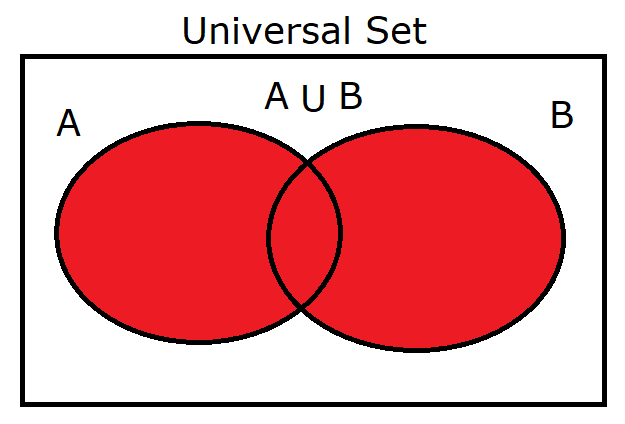(2.) $A \cap B$ Same as: $(A' \cup B')'$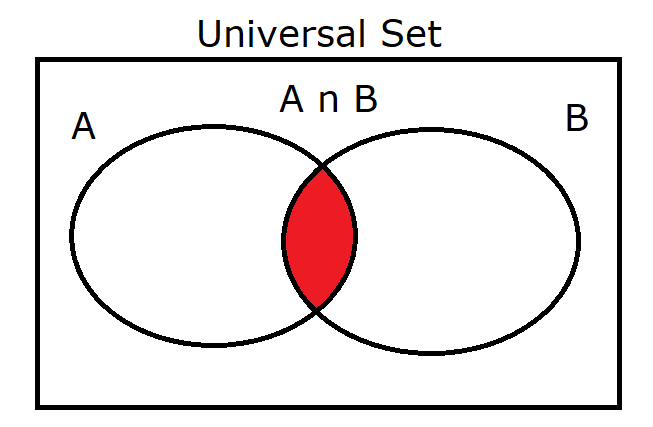(3.) $A' \cap B$ Same as: $(A \cup B')'$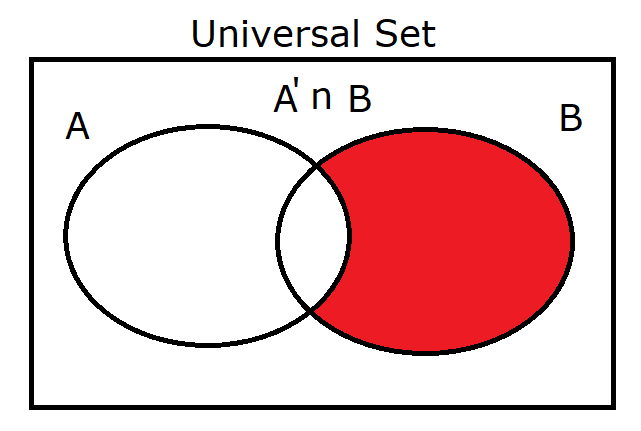(4.) $A \cap B'$ Same as: $(A' \cup B)'$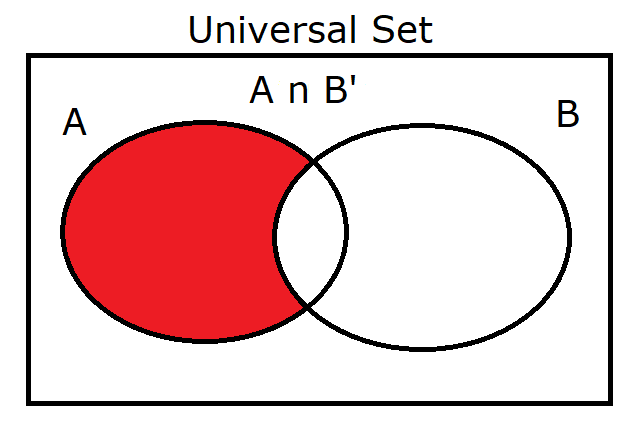(5.) $A' \cup B$ Same as: $(A \cap B')'$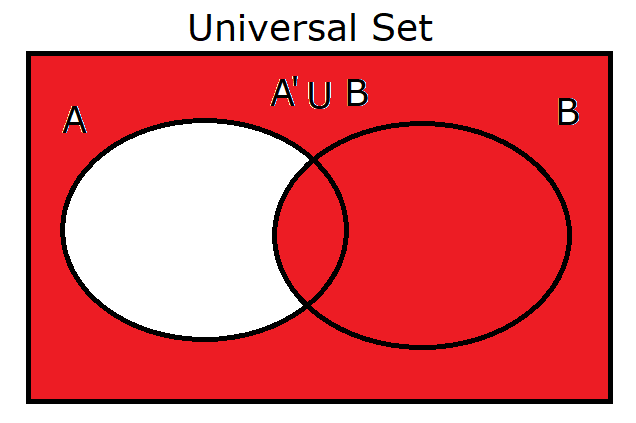(6.) $A \cup B'$ Same as: $(A' \cap B)'$(7.) $(A \cup B)'$ Same as: $A' \cap B'$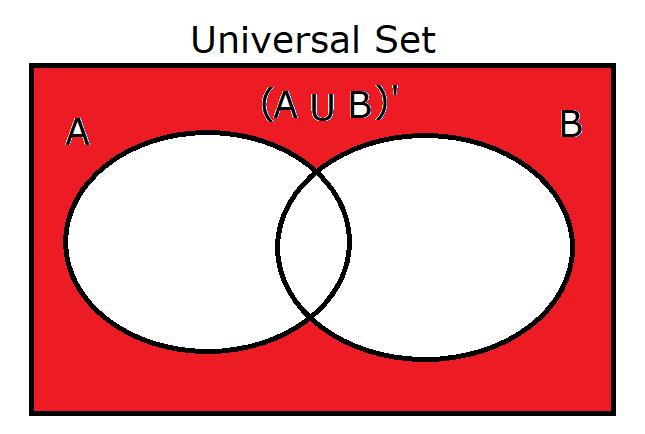(8.) $(A \cap B)'$ Same as: $A' \cup B'$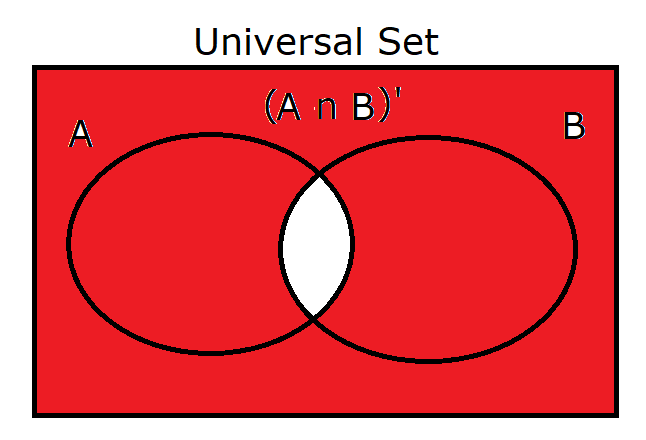(9.) $\mu$ Same as: $\phi'$ $A \cup A'$ $A \cup \mu$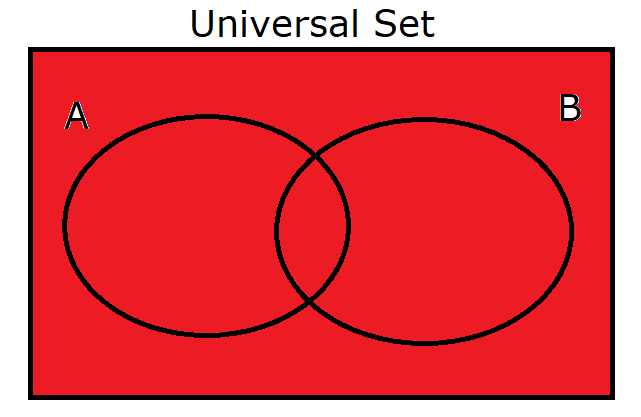(10.) $\phi$ Same as: $\mu'$ $A \cap A'$ $A \cap \phi$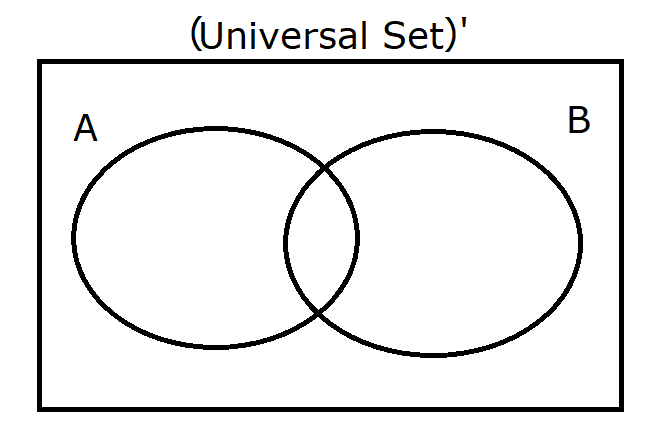(11.) $Disjoint\:\: Sets$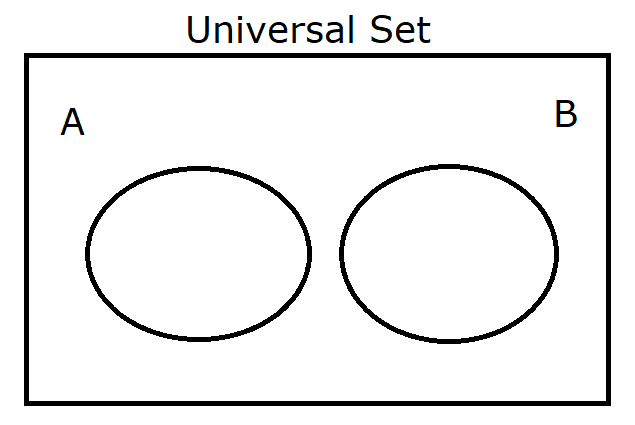(12.) $B \subset A$ Same as: $A \supset B$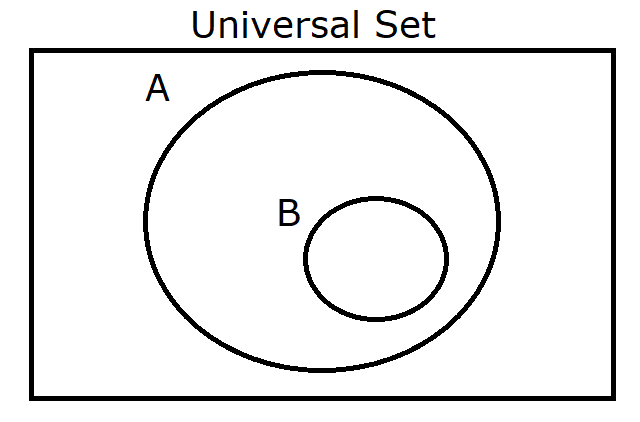(13.) $A$ Same as: $A \cup \phi$ $A \cap \mu$ $A \cup A$ $A \cap A$ $A \cup (A \cap B)$ $A \cap (A \cup B)$ $A''$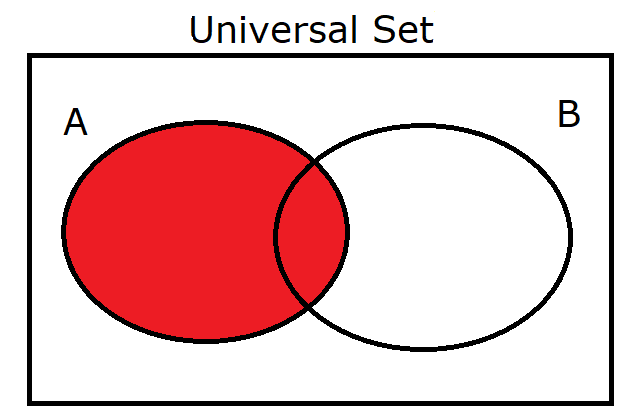(14.) $B$ Same as: $B \cup \phi$ $B \cap \mu$ $B \cup B$ $B \cap B$ $B \cup (A \cap B)$ $B \cap (A \cup B)$ $B''$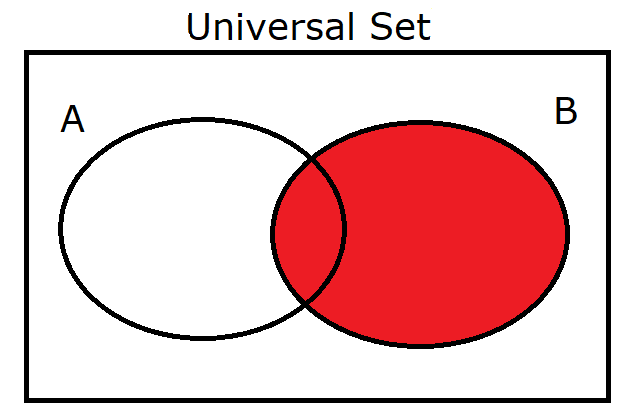(15.) $A'$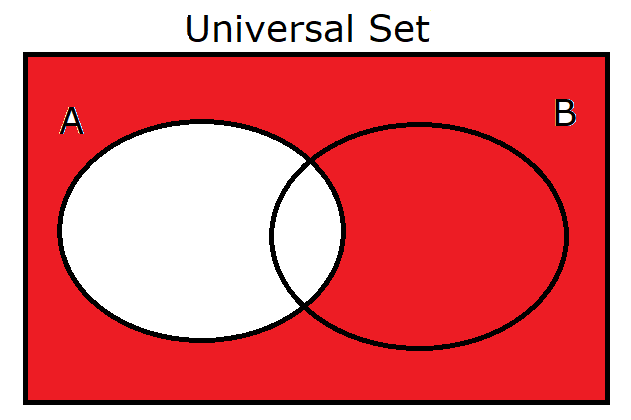(16.) $B'$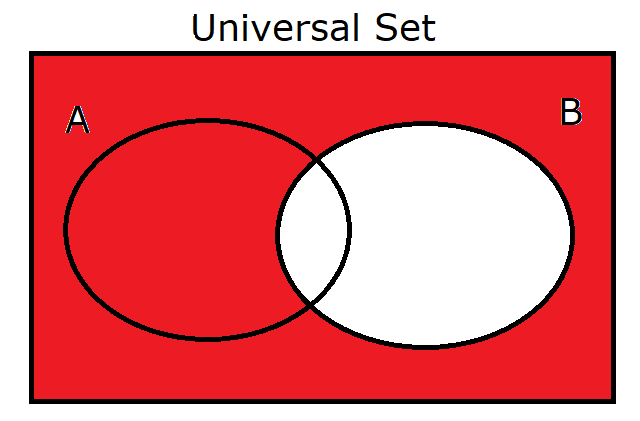### Venn Diagrams for Three Sets

Exercise 11
Draw the Venn diagrams, and shade the regions for these three sets.

Let us begin with a working copy.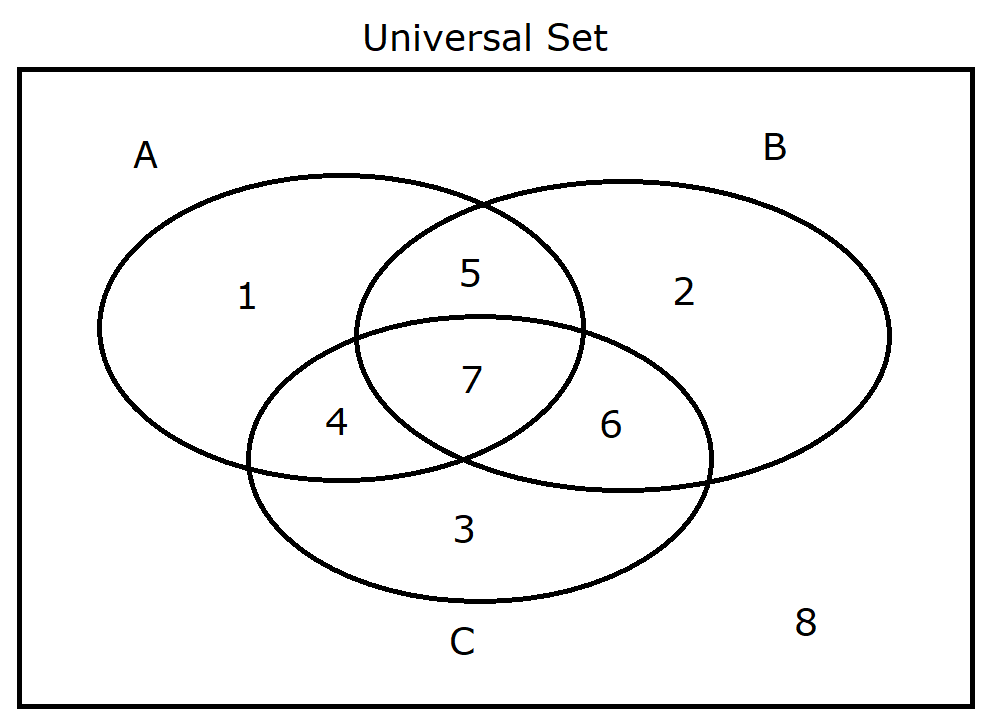$\mu = \{1, 2, 3, 4, 5, 6, 7, 8\} \\[3ex] A = \{1, 4, 5, 7\} \\[3ex] A' = \{2, 3, 6, 8\} \\[3ex] B = \{2, 5, 6, 7\} \\[3ex] B' = \{1, 3, 4, 8\} \\[3ex] C = \{3, 4, 6, 7\} \\[3ex] C' = \{1, 2, 5, 8\} \\[3ex] A \cup B \cup C = \{1, 2, 3, 4, 5, 6, 7\} \\[3ex] A \cap B \cap C = \{7\} \\[3ex] A' \cup B' \cup C' = \{1, 2, 3, 4, 5, 6, 8\} \\[3ex] A' \cap B' \cap C' = \{8\} \\[3ex] A \cup B = \{1, 2, 4, 5, 6, 7\} \\[3ex] A \cup C = \{1, 3, 4, 5, 6, 7\} \\[3ex] B \cup C = \{2, 3, 4, 5, 6, 7\} \\[3ex] A \cap B = \{5, 7\} \\[3ex] A \cap C = \{4, 7\} \\[3ex] B \cap C = \{6, 7\}$

(1.) $A$ OR $B$ OR $C$

$A \cup B \cup C = \{1, 2, 3, 4, 5, 6, 7\} \\[3ex] (A' \cap B' \cap C')' = \{1, 2, 3, 4, 5, 6, 7\}$

(2.) $A$ AND $B$ AND $C$

$A \cap B = \{5, 7\} \\[3ex] A \cap B \cap C = \{5, 7\} \cap \{3, 4, 6, 7\} = \{7\} \\[3ex] A \cap B \cap C = \{7\} \\[3ex] (A' \cup B' \cup C')' = \{7\}$

(3.) $A \cup (B \cap C)$

$B \cap C = \{6, 7\} \\[3ex] A \cup (B \cap C) = \{1, 4, 5, 7\} \cup \{6, 7\} \\[3ex] A \cup (B \cap C) = \{1, 4, 5, 6, 7\} \\[3ex] (A \cup B) \cap (A \cup C) = \{1, 4, 5, 6, 7\}$

(4.) $B \cup (A \cap C)$

$A \cap C = \{4, 7\} \\[3ex] B \cup (A \cap C) = \{2, 5, 6, 7\} \cup \{4, 7\} \\[3ex] A \cup (B \cap C) = \{2, 4, 5, 6, 7\} \\[3ex] (B \cup A) \cap (B \cup C) = \{2, 4, 5, 6, 7\}$

(5.) $C \cup (A \cap B)$

$A \cap B = \{5, 7\} \\[3ex] C \cup (A \cap B) = \{3, 4, 6, 7\} \cup \{5, 7\} \\[3ex] A \cup (B \cap C) = \{3, 4, 5, 6, 7\} \\[3ex] (C \cup A) \cap (C \cup B) = \{3, 4, 5, 6, 7\}$

(6.) $A \cup B \cap C$

$A \cup B = \{1, 2, 4, 5, 6, 7\} \\[3ex] A \cup B \cap C = \{1, 2, 4, 5, 6, 7\} \cap \{3, 4, 6, 7\} \\[3ex] A \cup B \cap C = \{4, 6, 7\} \\[3ex] (A' \cap B' \cup C')' = \{4, 6, 7\}$

(7.) $B \cup C \cap A$

$B \cup C = \{2, 3, 4, 5, 6, 7\} \\[3ex] A \cup B \cap C = \{2, 3, 4, 5, 6, 7\} \cap \{1, 4, 5, 7\} \\[3ex] A \cup B \cap C = \{4, 5, 7\} \\[3ex] (B' \cap C' \cup A')' = \{4, 5, 7\}$

(8.) $A \cup C \cap B$

$A \cup C = \{1, 3, 4, 5, 6, 7\} \\[3ex] A \cup C \cap B = \{1, 3, 4, 5, 6, 7\} \cap \{2, 5, 6, 7\} \\[3ex] A \cup C \cap B = \{5, 6, 7\} \\[3ex] (A' \cap C' \cup B')' = \{5, 6, 7\}$

(9.) $A \cup B \cup C'$

$A \cup B = \{1, 2, 4, 5, 6, 7\} \\[3ex] A \cup B \cup C' = \{1, 2, 4, 5, 6, 7\} \cup \{1, 2, 5, 8\} \\[3ex] A \cup B \cup C' = \{1, 2, 4, 5, 6, 7, 8\} \\[3ex] (A' \cap B' \cap C)' = \{1, 2, 4, 5, 6, 7, 8\}$

(10.) $A \cup B' \cup C$

$A \cup B' = \{1, 3, 4, 5, 7, 8\} \\[3ex] A \cup B' \cup C = \{1, 3, 4, 5, 7, 8\} \cup \{3, 4, 6, 7\} \\[3ex] A \cup B' \cup C = \{1, 3, 4, 5, 6, 7, 8\} \\[3ex] (A' \cap B \cap C')' = \{1, 3, 4, 5, 6, 7, 8\}$

(11.) $A' \cup B \cup C$

$A' \cup B = \{2, 3, 5, 6, 7, 8\} \\[3ex] A' \cup B \cup C = \{2, 3, 5, 6, 7, 8\} \cup \{3, 4, 6, 7\} \\[3ex] A' \cup B \cup C = \{2, 3, 4, 5, 6, 7, 8\} \\[3ex] (A \cap B' \cap C')' = \{2, 3, 4, 5, 6, 7, 8\}$

(12.) $A$ OR $B$ but NOT $C$

$A \cup B \cap C' = \{1, 2, 5\} \\[3ex] (A' \cap B' \cup C)' = \{1, 2, 5\}$

(13.) $A$ OR $C$ but NOT $B$

$A \cup C \cap B' = \{1, 3, 4\} \\[3ex] (A' \cap C' \cup B)' = \{1, 3, 4\}$

(14.) $B$ OR $C$ but NOT $A$

$B \cup C \cap A' = \{2, 3, 6\} \\[3ex] (B' \cap C' \cup A)' = \{2, 3, 6\}$

(15.) $A$ AND $B$ but NOT $C$
$A$ AND $B$ ONLY

$A \cap B \cap C' = \{5\} \\[3ex] (A' \cup B' \cup C)' = \{5\}$

(16.) $A$ AND $C$ but NOT $B$
$A$ AND $C$ ONLY

$A \cap B' \cap C = \{4\} \\[3ex] (A' \cup B \cup C')' = \{4\}$

(17.) $B$ AND $C$ but NOT $A$
$B$ AND $C$ ONLY

$A' \cap B \cap C = \{6\} \\[3ex] (A \cup B' \cup C')' = \{6\}$

(18.) $A$ ONLY
$A \cap B' \cap C' = \{1\}$
$(A' \cup B \cup C)' = \{1\}$

(19.) $B$ ONLY

$A' \cap B \cap C' = \{2\} \\[3ex] (A \cup B' \cup C)' = \{2\}$

(20.) $C$ ONLY

$A' \cap B' \cap C = \{3\} \\[3ex] (A \cup B \cup C')' = \{3\}$

 (1.) $A \cup B \cup C$ Same as: $(A' \cap B' \cap C')'$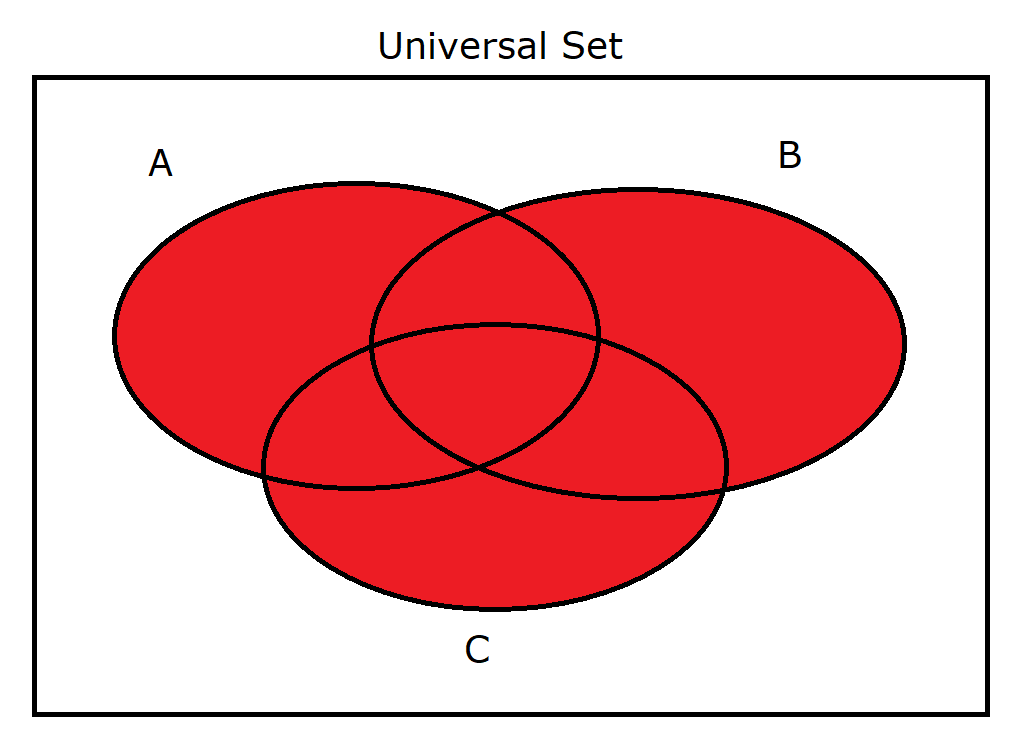(2.) $A \cap B \cap C$ Same as: $(A' \cup B' \cup C')'$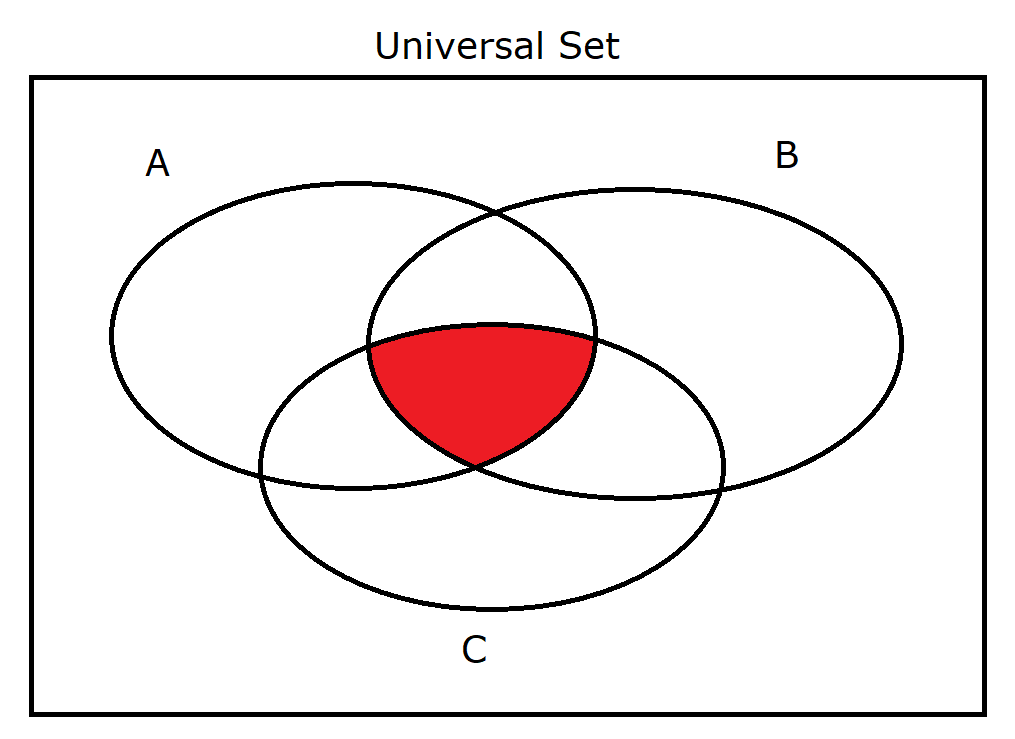(3.) $A \cup (B \cap C)$ Same as: $(A \cup B) \cap (A \cup C)$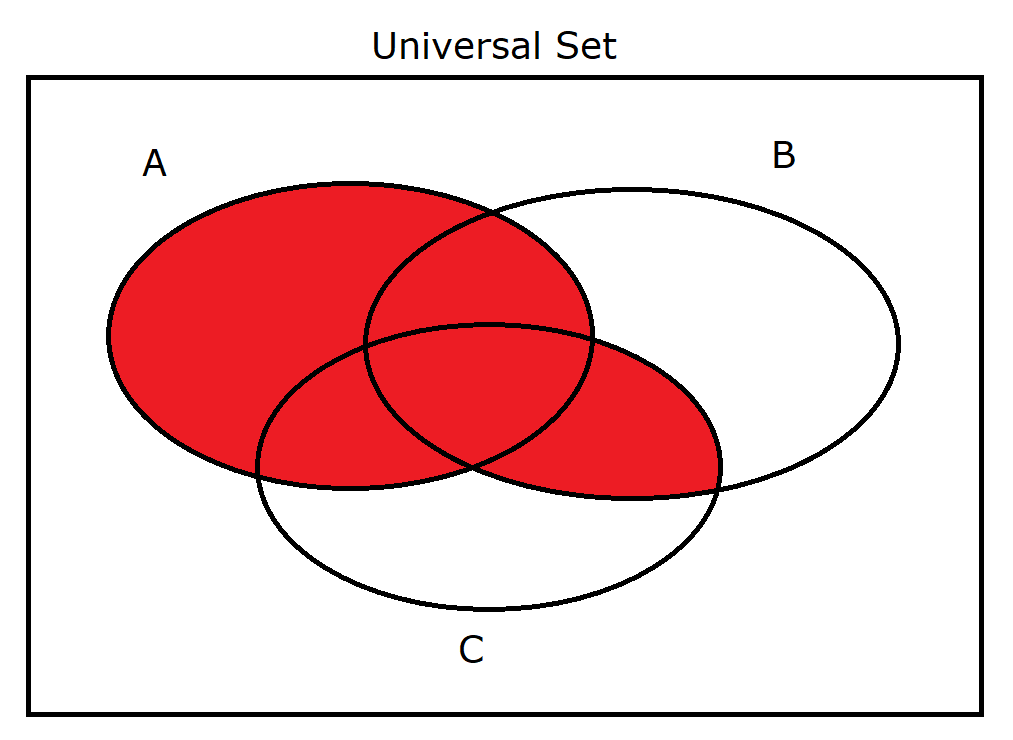(4.) $B \cup (A \cap C)$ Same as: $(B \cup A) \cap (B \cup C)$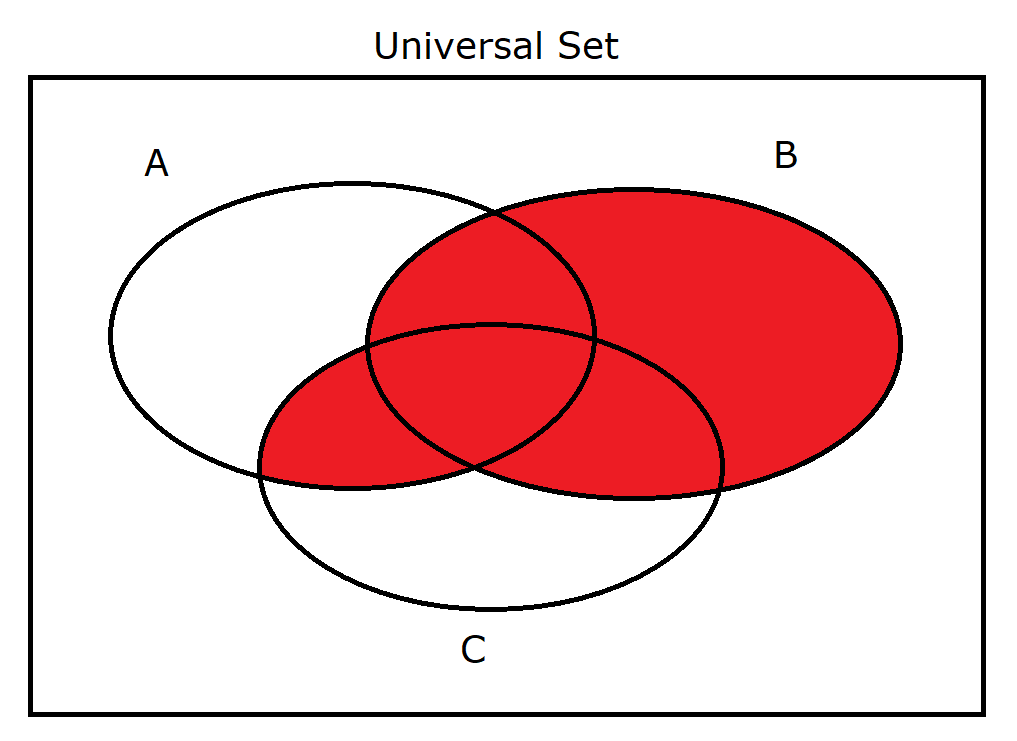(5.) $C \cup (A \cap B)$ Same as: $(C \cup A) \cap (C \cup B)$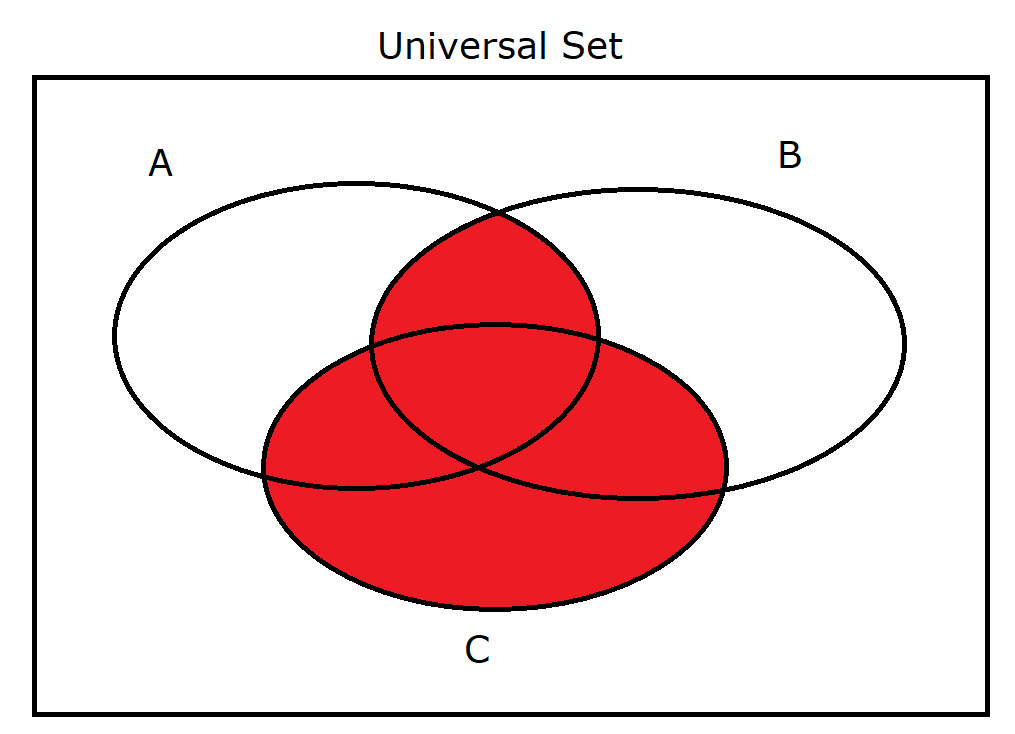(6.) $A \cup B \cap C$ Same as: $(A' \cap B' \cup C')'$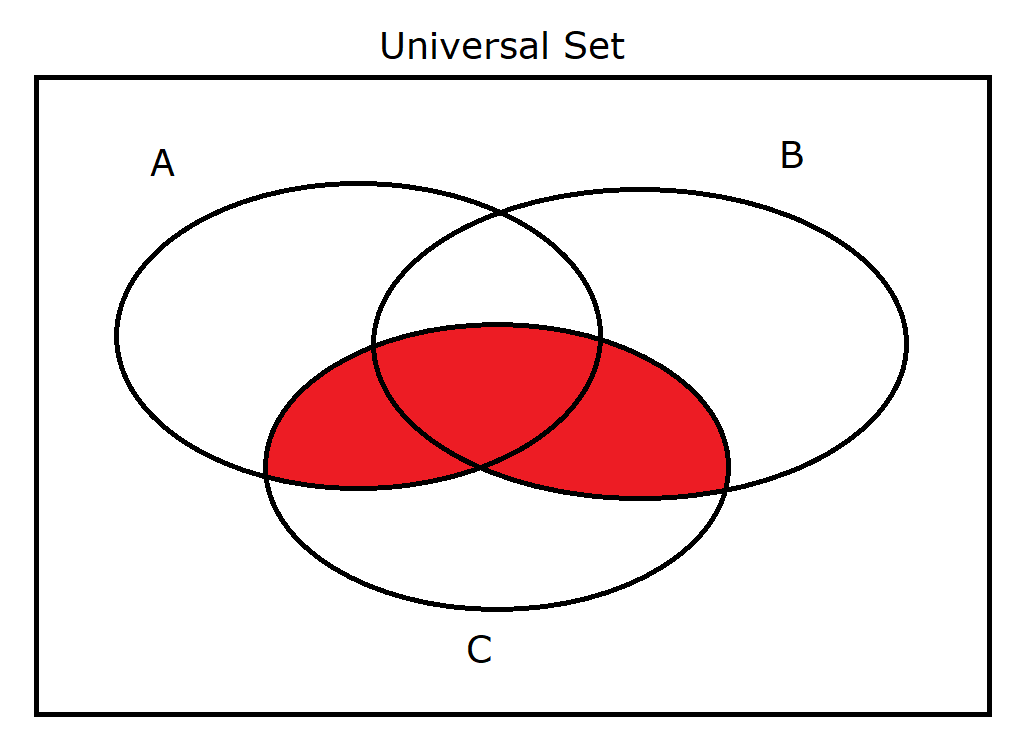(7.) $B \cup C \cap A$ Same as: $(B' \cap C' \cup A')'$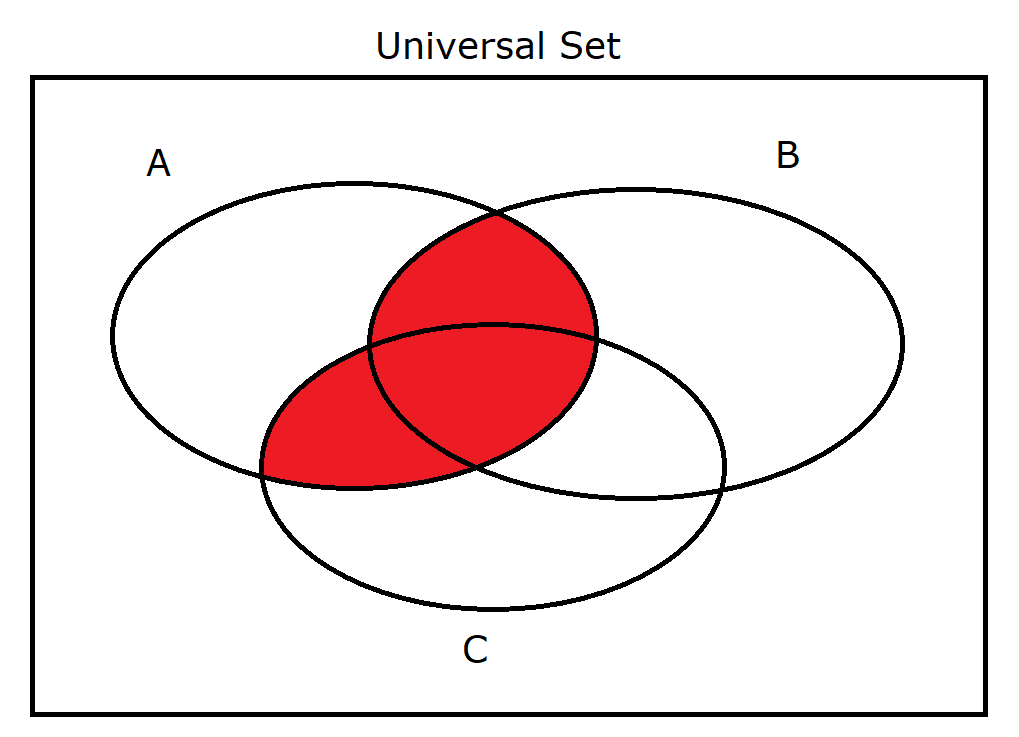(8.) $A \cup C \cap B$ Same as: $(A' \cap C' \cup B')'$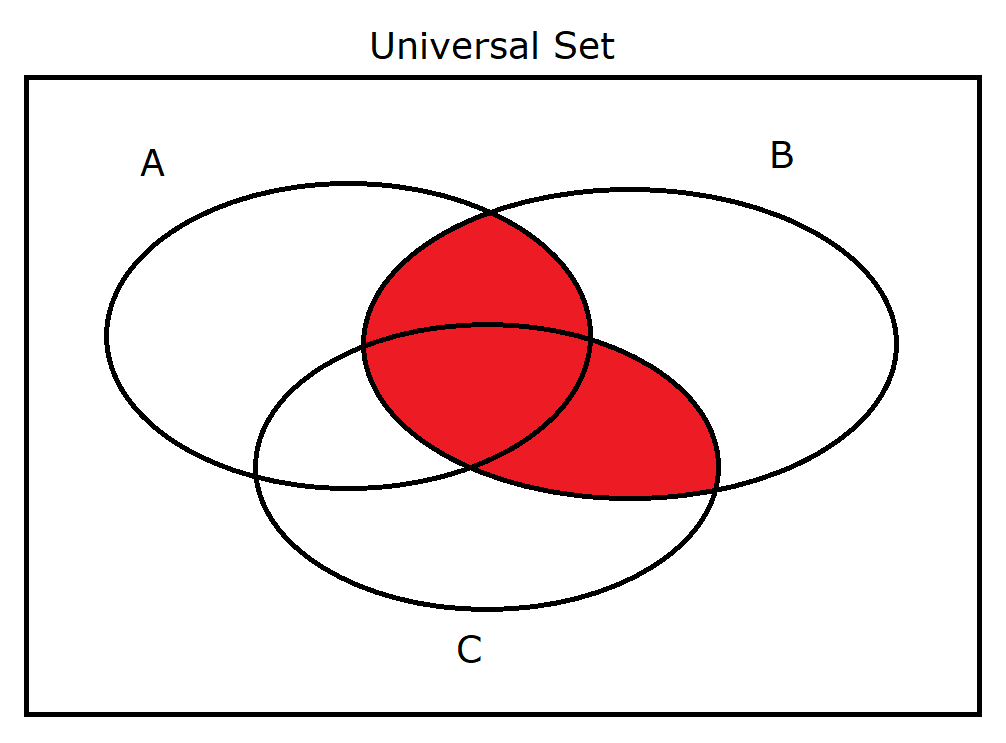(9.) $A \cup B \cup C'$ Same as: $(A' \cap B' \cap C)'$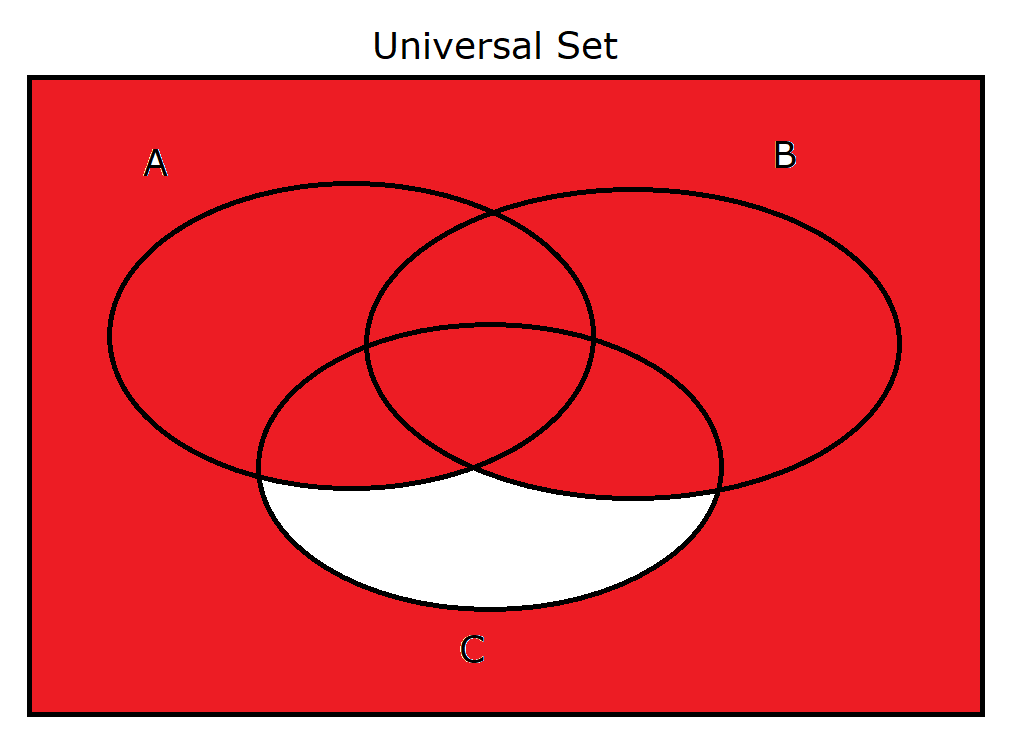(10.) $A \cup B' \cup C$ Same as: $(A' \cap B \cap C')'$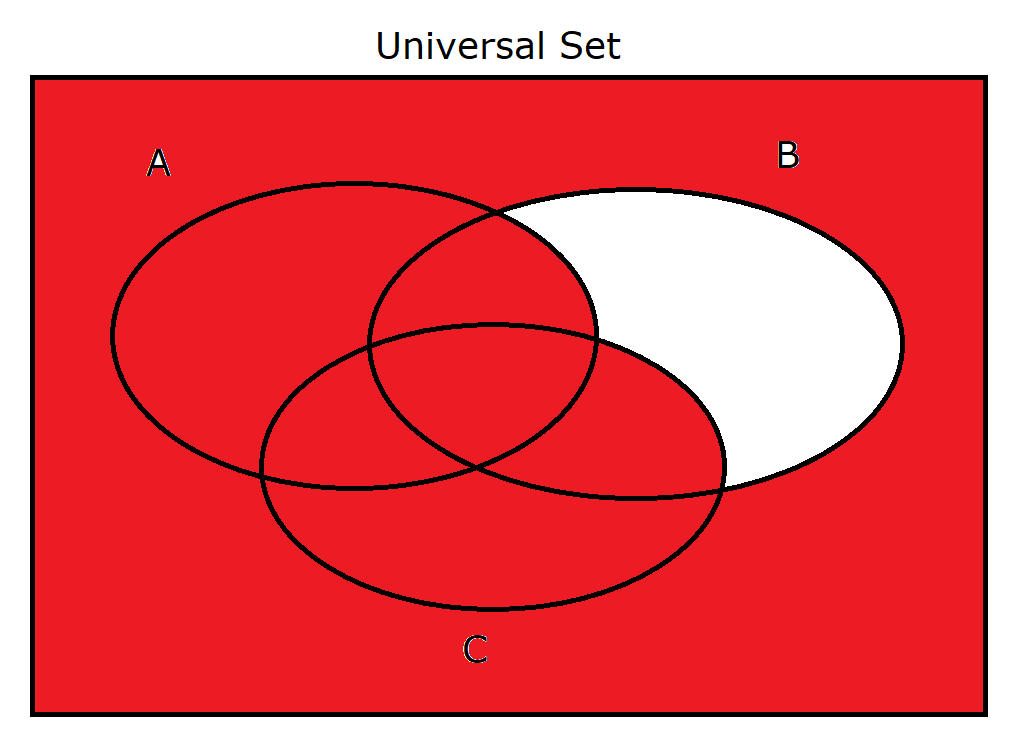(11.) $A' \cup B \cup C$ Same as: $(A \cap B' \cap C')'$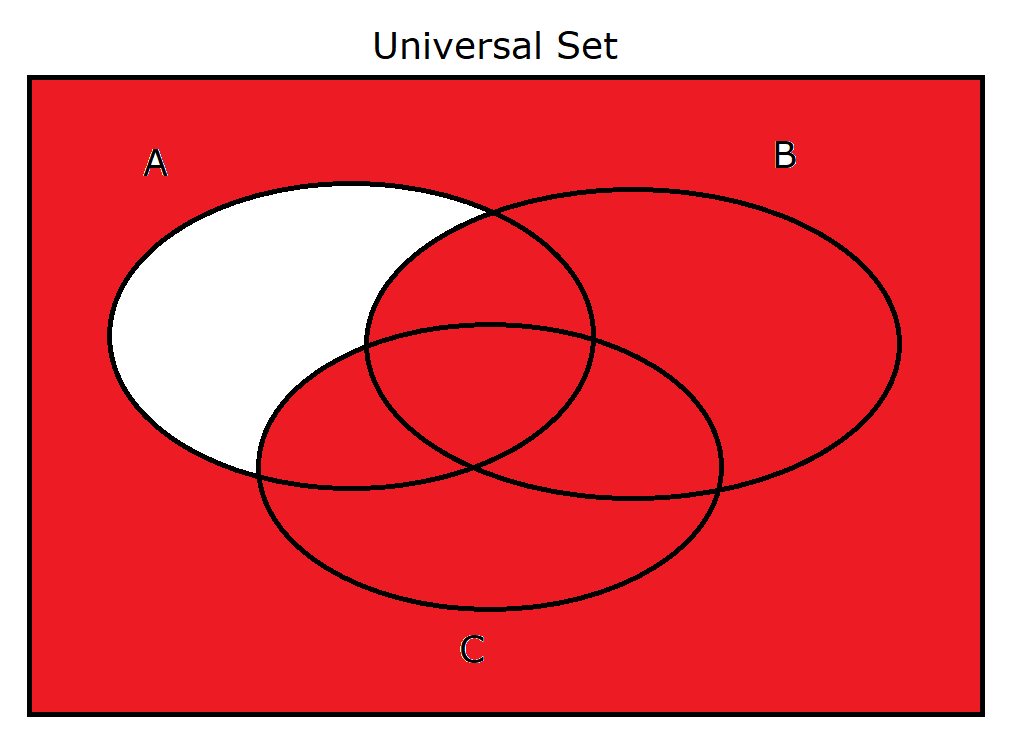(12.) $A \cup B \cap C'$ Same as: $(A' \cap B' \cup C)'$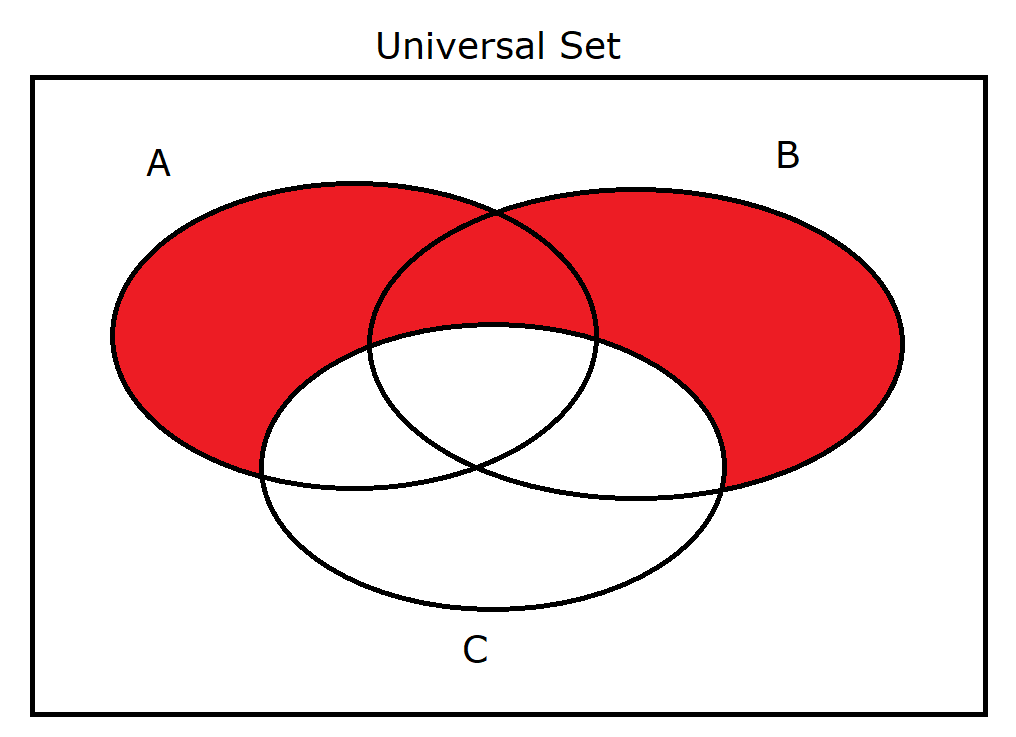(13.) $A \cup C \cap B'$ Same as: $(A' \cap C' \cup B)'$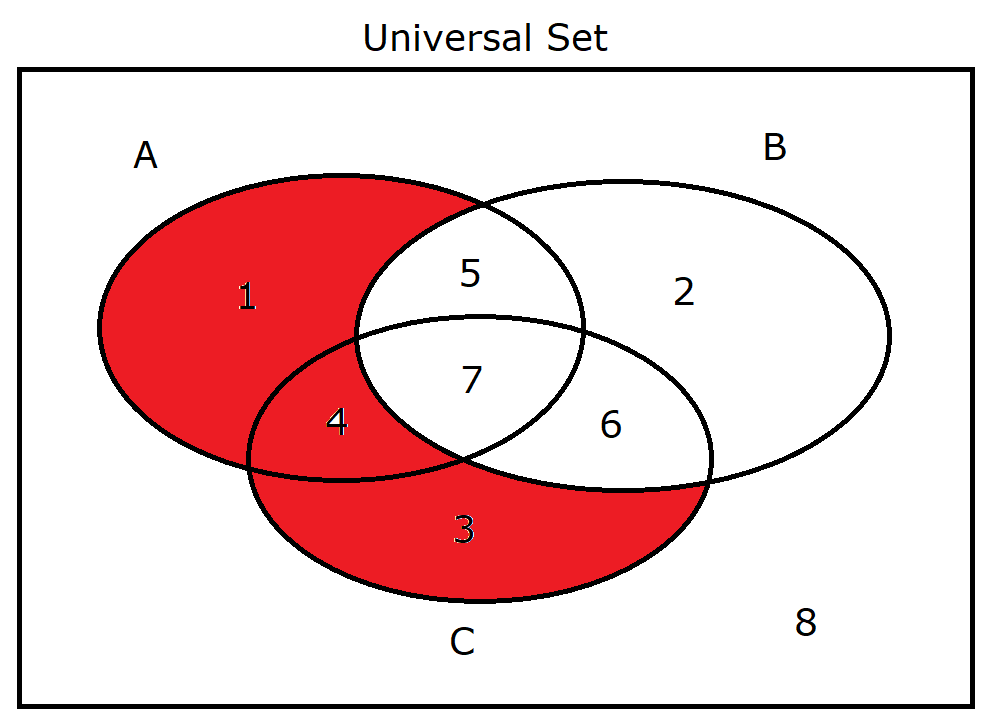(14.) $B \cup C \cap A'$ Same as: $(B' \cap C' \cup A)'$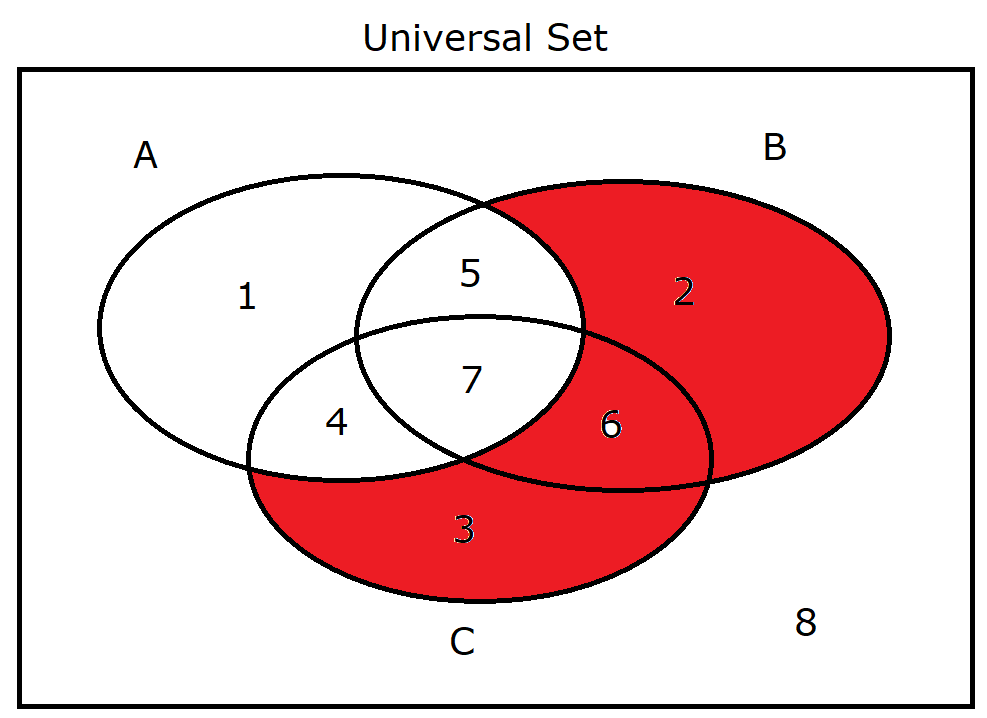(15.) $A \cap B \cap C'$ Same as: $(A' \cup B' \cup C)'$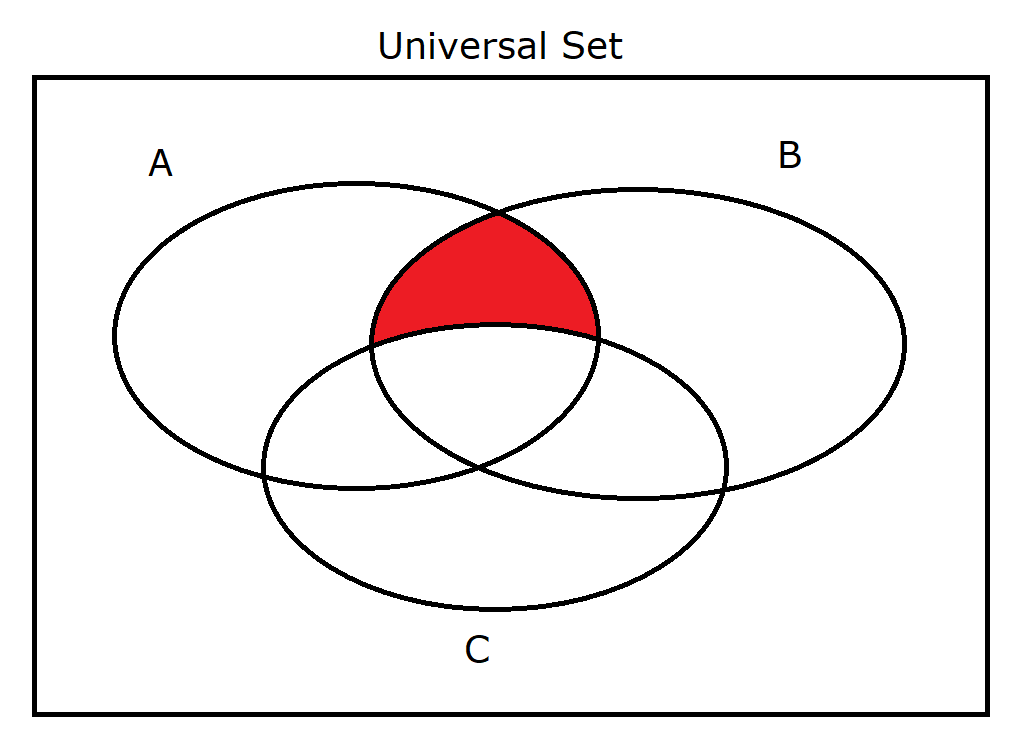(16.) $A \cap B' \cap C$ Same as: $(A' \cup B \cup C')'$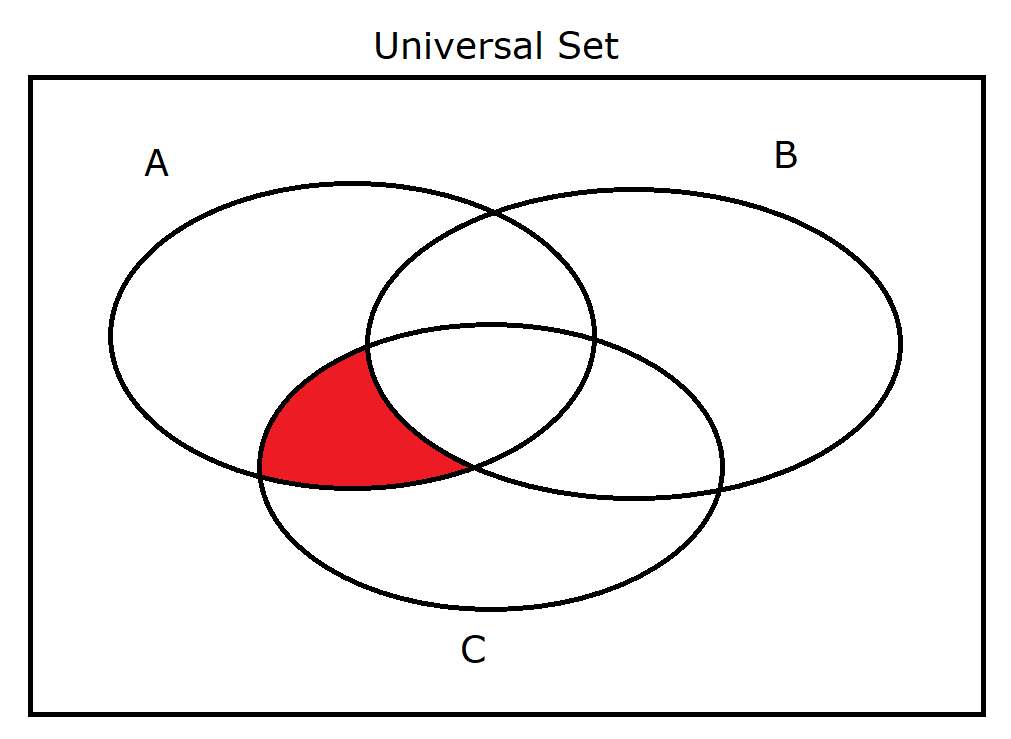(17.) $A' \cap B \cap C$ Same as: $(A \cup B' \cup C')'$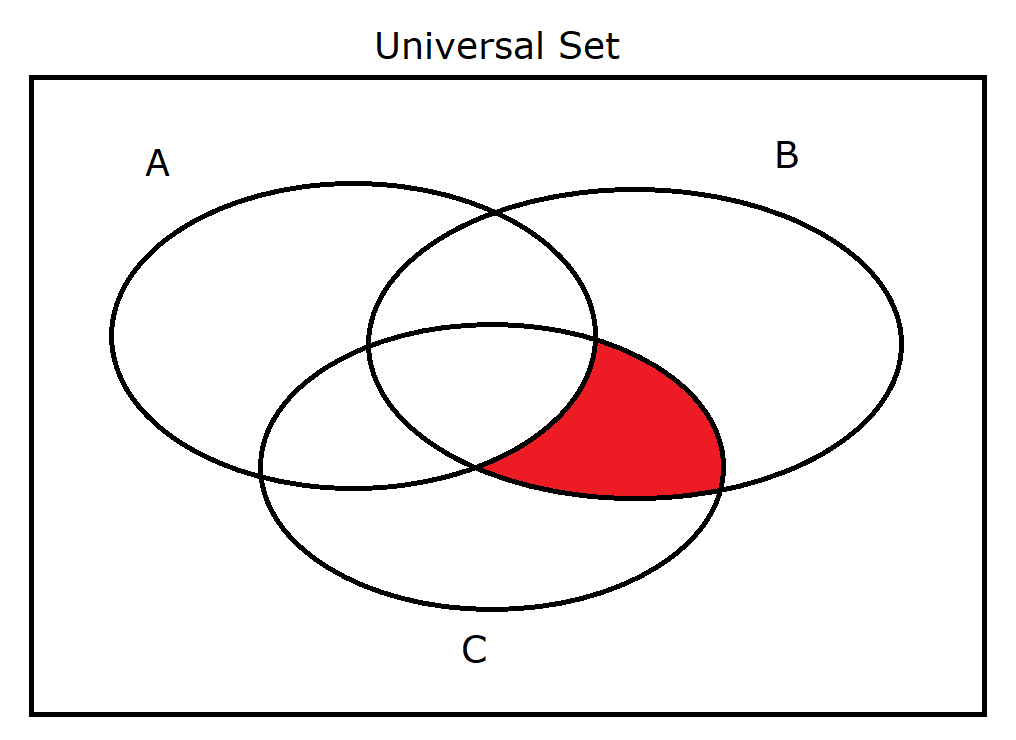(18.) $A \cap B' \cap C'$ Same as: $(A' \cup B \cup C)'$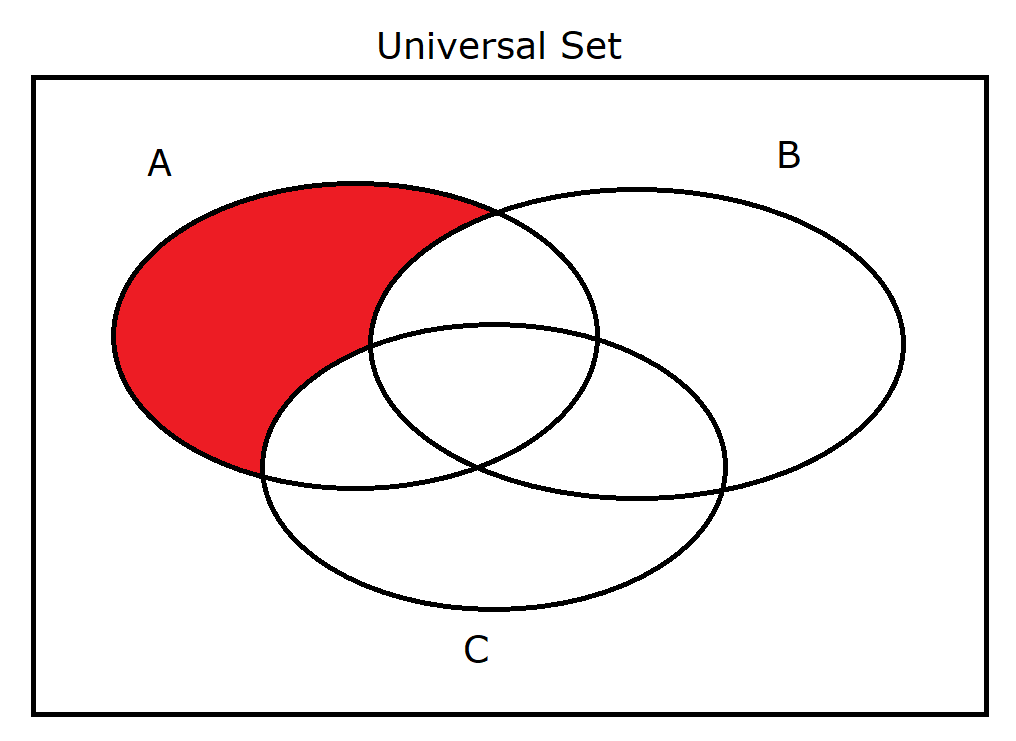(19.) $A' \cap B \cap C'$ Same as: $(A \cup B' \cup C)'$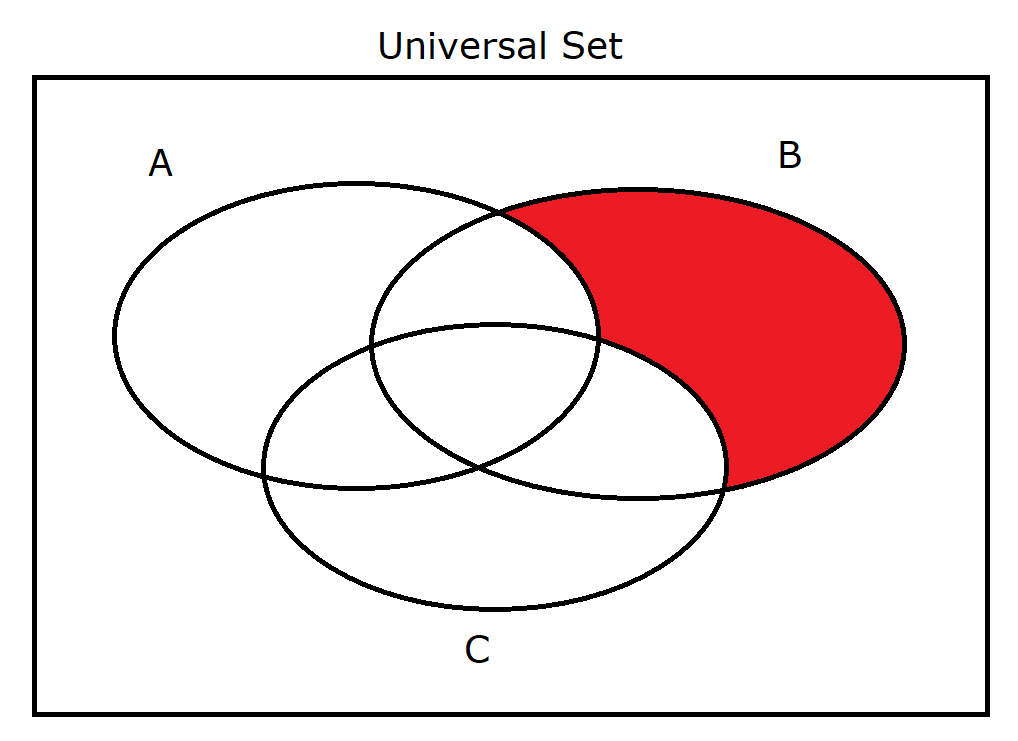(20.) $A' \cap B' \cap C$ Same as: $(A \cup B \cup C')'$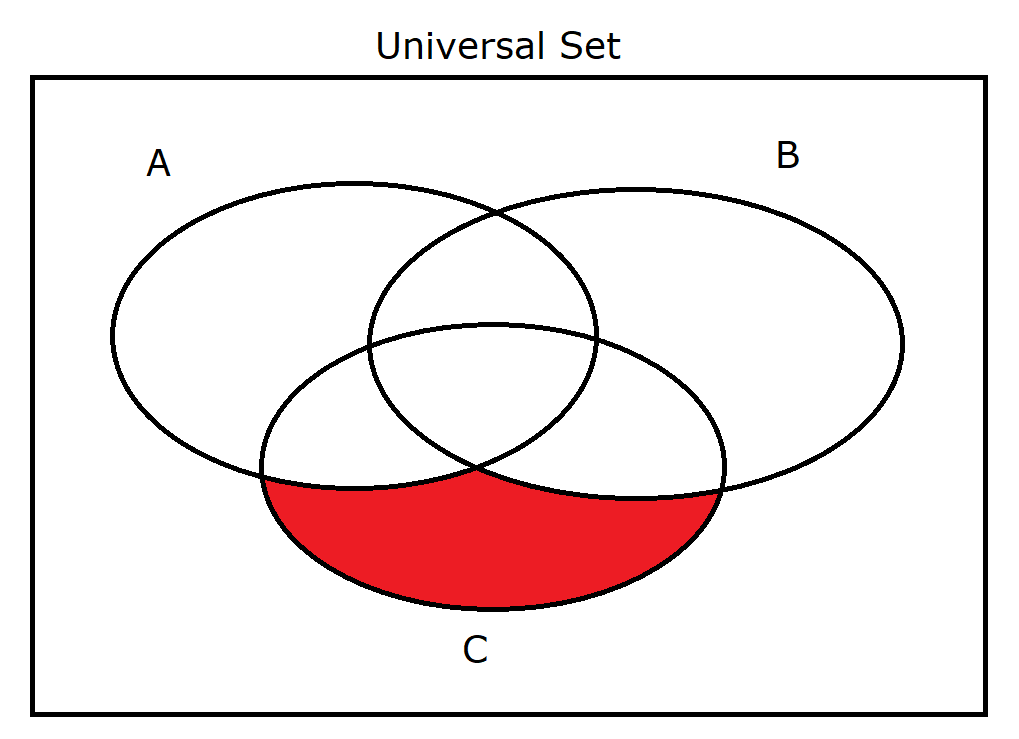(21.) $A' \cap B' \cap C'$ Same as: $(A \cup B \cup C)'$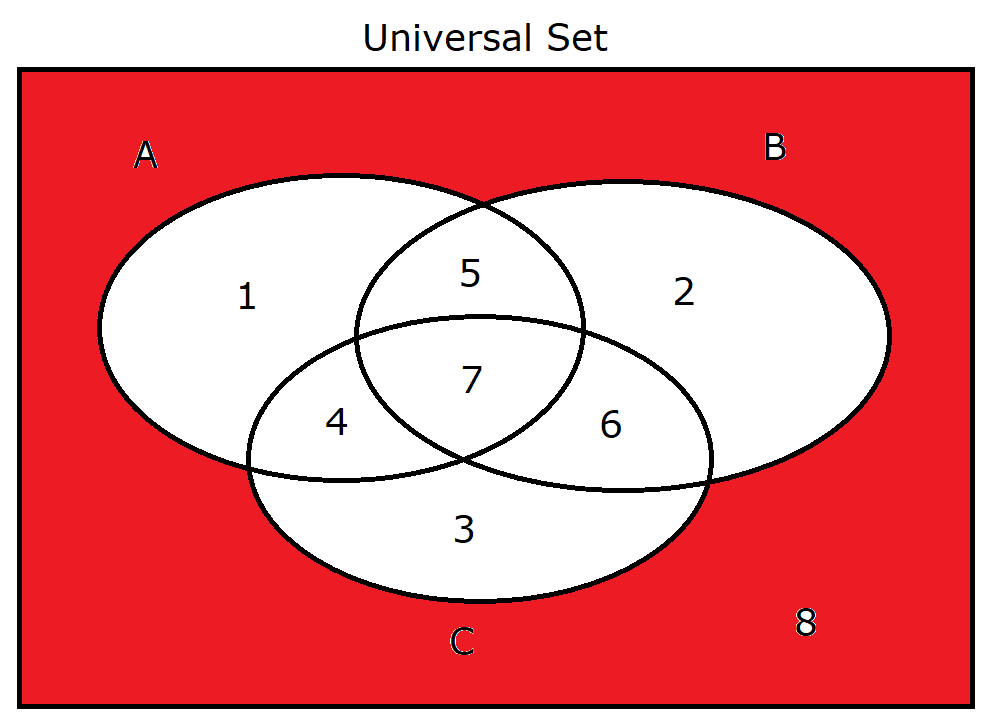(22.) $A$ Same as: $A \cup \phi$ $A \cap \mu$ $A \cup A$ $A \cap A$ $A''$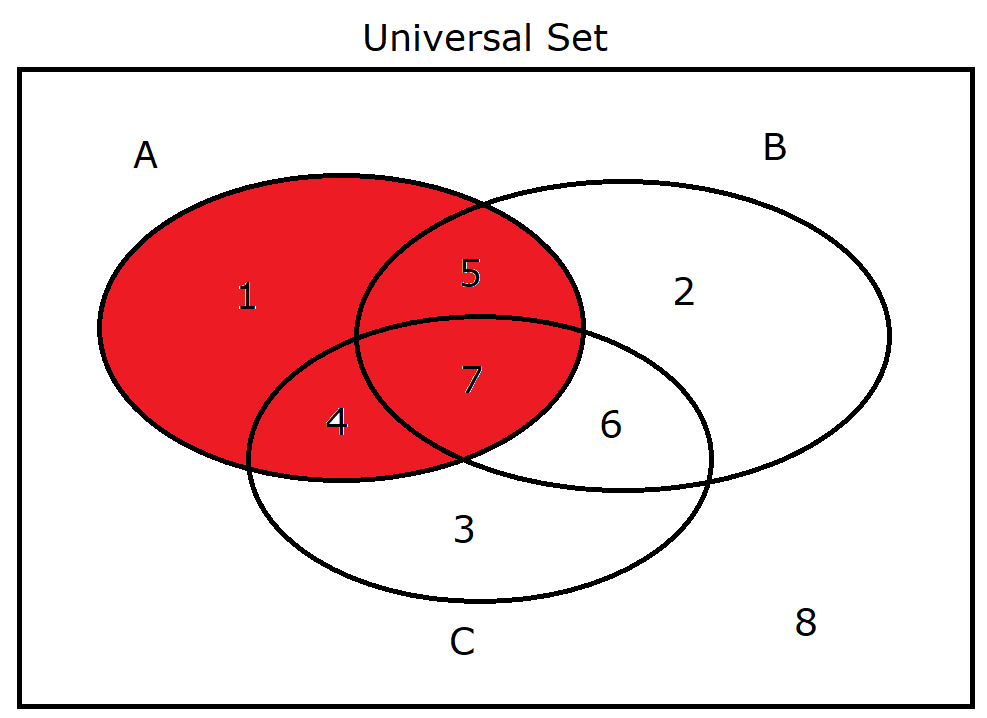(23.) $B$ Same as: $B \cup \phi$ $B \cap \mu$ $B \cup B$ $B \cap B$ $B''$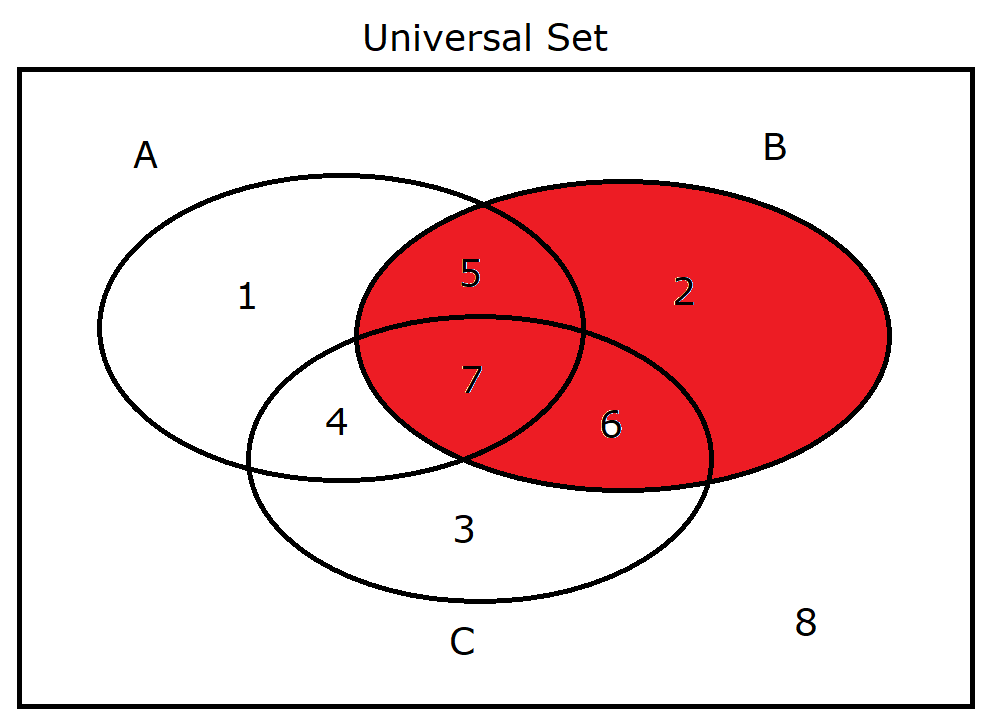(24.) $C$ Same as: $C \cup \phi$ $C \cap \mu$ $C \cup C$ $C \cap C$ $C''$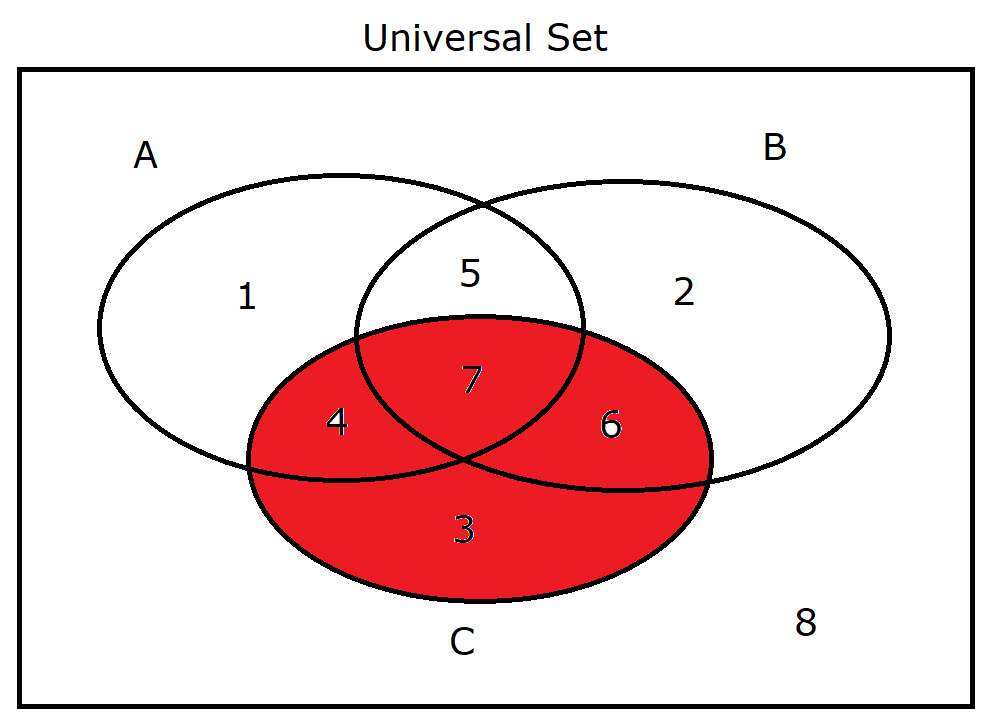(25.) $A'$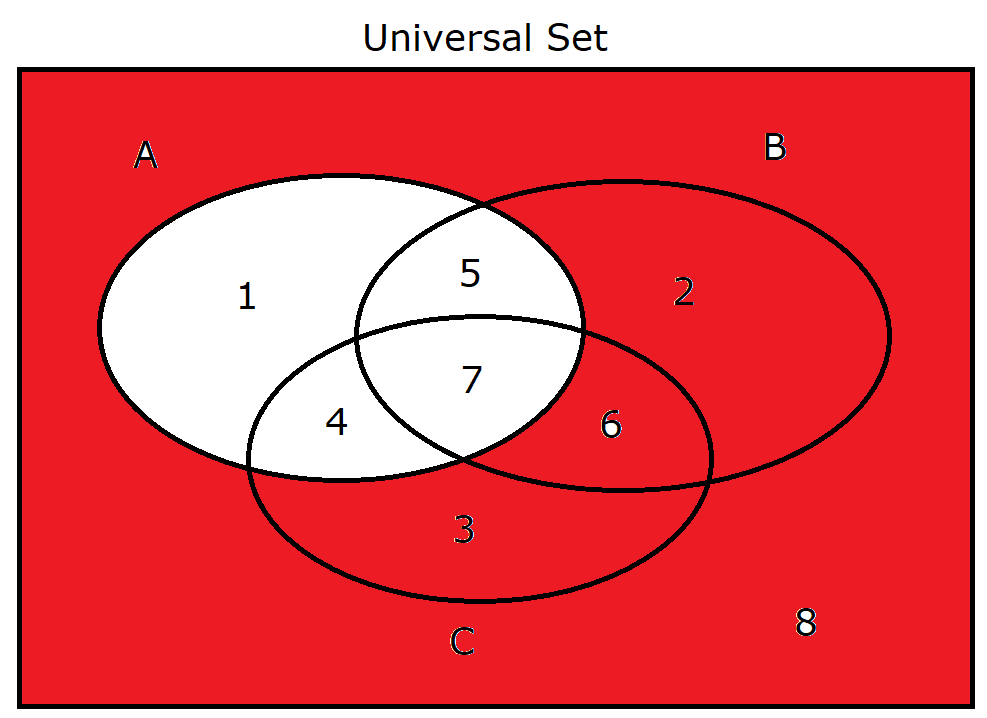(26.) $B'$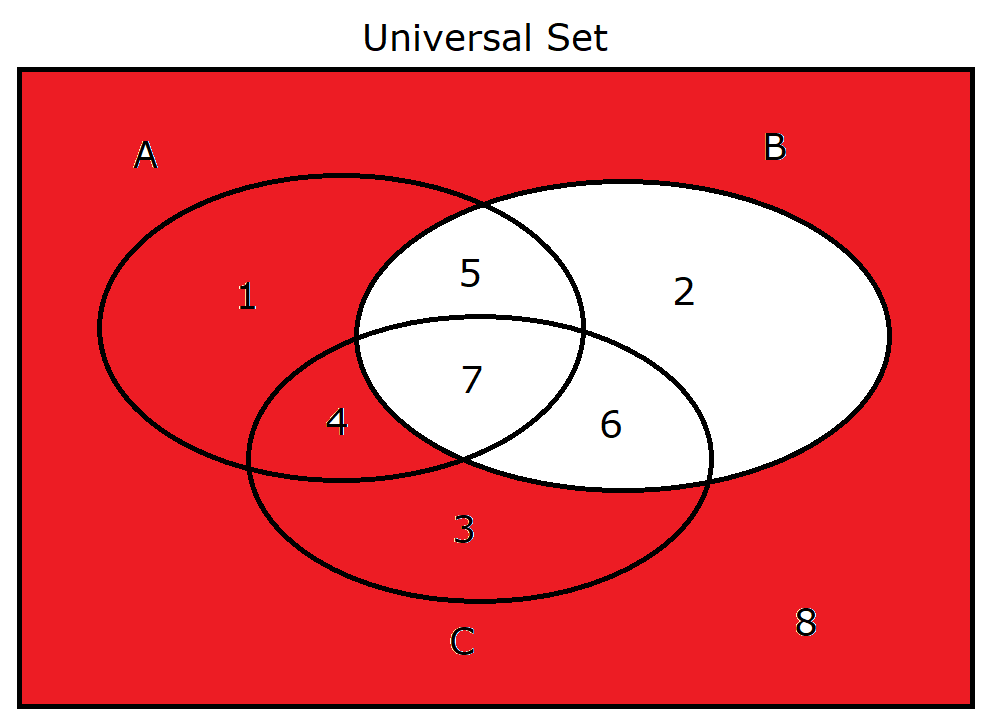(27.) $C'$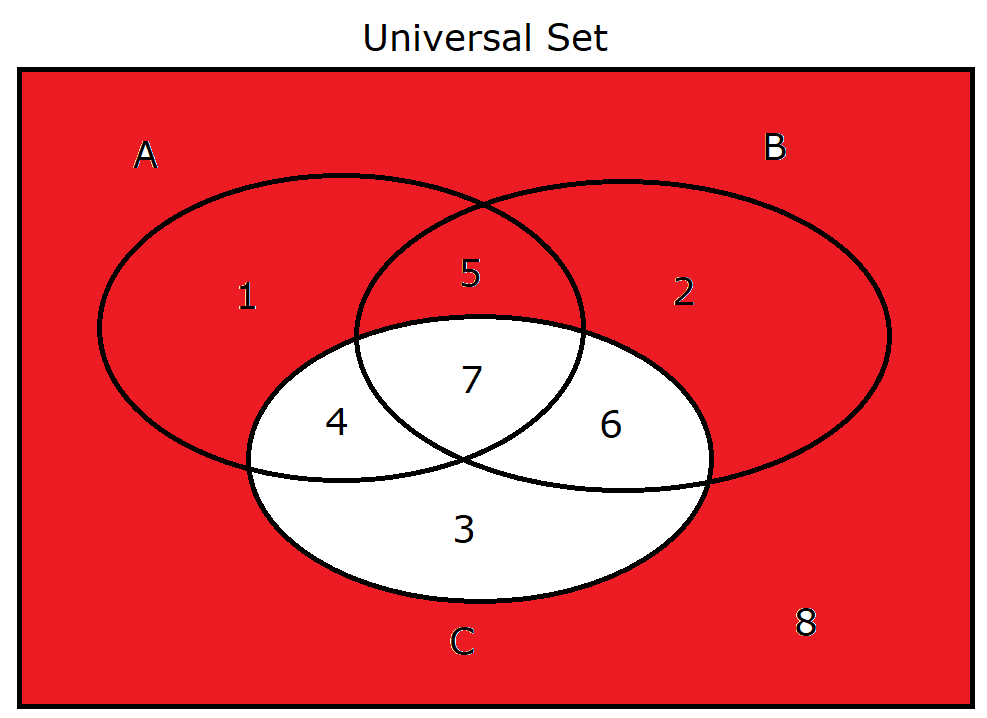(28.) $A \cup B' \cap C$ Same as: $(A' \cap B \cup C')'$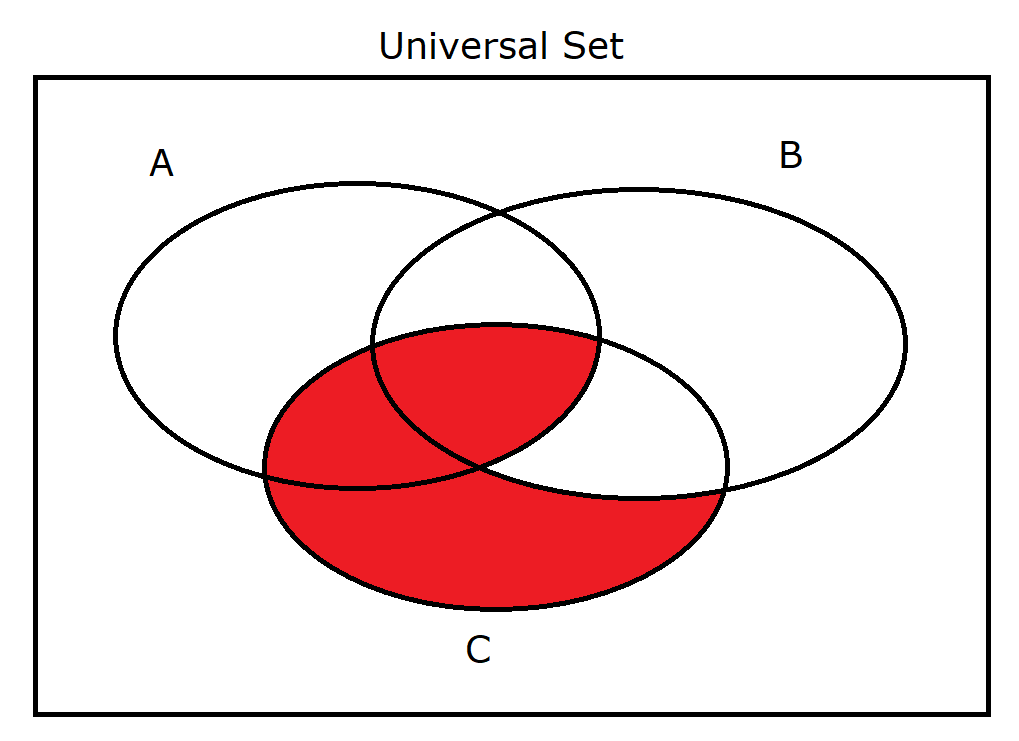(29.) $A' \cup B \cap C$ Same as: $(A \cap B' \cup C')'$